# On the Hardness of Module Learning with Errors with Short Distributions

## Abstract

The Module Learning With Errors ($$\text {M-LWE}$$) problem is a core computational assumption of lattice-based cryptography which offers an interesting trade-off between guaranteed security and concrete efficiency. The problem is parameterized by a secret distribution as well as an error distribution. There is a gap between the choices of those distributions for theoretical hardness results (standard formulation of $$\text {M-LWE}$$, i.e., uniform secret modulo q and Gaussian error) and practical schemes (small bounded secret and error). In this work, we make progress toward narrowing this gap. More precisely, we prove that $$\text {M-LWE}$$ with uniform $$\eta$$-bounded secret for any $$1 \le \eta \ll q$$ and Gaussian error, in both its search and decision variants, is at least as hard as the standard formulation of $$\text {M-LWE}$$, provided that the module rank d is at least logarithmic in the ring degree n. We also prove that the search version of $$\text {M-LWE}$$ with large uniform secret and uniform $$\eta$$-bounded error is at least as hard as the standard $$\text {M-LWE}$$ problem, if the number of samples m is close to the module rank d and with further restrictions on $$\eta$$. The latter result can be extended to provide the hardness of search $$\text {M-LWE}$$ with uniform $$\eta$$-bounded secret and error under specific parameter conditions. Overall, the results apply to all cyclotomic fields, but most of the intermediate results are proven in more general number fields.

This is a preview of subscription content, access via your institution.

We’re sorry, something doesn't seem to be working properly.

1. Note that at the time of writing, the paper by Lin et al. is only accessible on ePrint and has not yet been peer-reviewed.

2. Setting $$\eta = 1$$ gives ternary secrets instead of binary. We, however, observe that the parameters covered by the reductions for $$\eta =1$$ in the centered representation match those of [12, 13], and it has the upside of a larger secret space. This leads to smaller ranks d by a factor of $$\log _2 3$$.

3. We may sometimes call this class of fields “monogenic fields,” but we note that rigorously a monogenic number field is $$K = {\mathbb {Q}}(\zeta )$$ for which $$R = {\mathbb {Z}}[\zeta ']$$ for a possibly different $$\zeta '$$.

4. In the degenerate case, the probability density function cannot be defined with respect to the Lebesgue measure as $$\varvec{{\Sigma }}$$ is not invertible. Standard facts on non-singular Gaussian distributions can, however, be extended to the degenerate case by using the characteristic function which always exists and equals $$\varphi _{\textbf{x}}({\textbf{t}}) = {\mathbb {E}}_{\textbf{x}}[\exp (i{\textbf{t}}^T{\textbf{x}})] = \exp (i{\textbf{c}}^T{\textbf{t}}- \pi {\textbf{t}}^T\varvec{{\Sigma }}{\textbf{t}})$$. In particular, one can easily show that the sum of two independent (potentially degenerate) Gaussians of covariance $$\varvec{{\Sigma }}_1, \varvec{{\Sigma }}_2$$ is a (potentially degenerate) Gaussian of covariance $$\varvec{{\Sigma }}_1 + \varvec{{\Sigma }}_2$$, as needed in this paper. We also note that one can still define a density, but with respect to a degenerate measure as $$D_{{\textbf{c}}, \sqrt{\varvec{{\Sigma }}}}({\textbf{x}}) = (\det ^+(\varvec{{\Sigma }}))^{-1/2}\exp (-\pi ({\textbf{x}}- {\textbf{c}})^T\varvec{{\Sigma }}^{+}({\textbf{x}}- {\textbf{c}}))$$, where $$\varvec{{\Sigma }}^+$$ is the Moore–Penrose pseudo-inverse, and $$\det ^+$$ the pseudo-determinant.

5. If q is inert, then $$R_q$$ is a field, and therefore $$Z(R_q) = \{0\}$$, meaning that there does not exist rs satisfying the conditions.

## References

1. M.R. Albrecht, A. Deo. Large modulus ring-lwe $$\ge$$ module-lwe. In ASIACRYPT (1), volume 10624 of Lecture Notes in Computer Science, (Springer, 2017) pp. 267–296

2. M.R. Albrecht, A. Deo. Large modulus ring-lwe $$>=$$ module-lwe. IACR Cryptol. ePrint Arch., (2017) p. 612

3. Martin R. Albrecht, Carlos Cid, Jean-Charles Faugère, Robert Fitzpatrick, and Ludovic Perret. Algebraic algorithms for LWE problems. ACM Commun. Comput. Algebra, 49(2):62, 2015.

4. J. Alperin-Sheriff, D. Apon. Dimension-preserving reductions from LWE to LWR. IACR Cryptol. ePrint Arch, 2016, p. 589

5. B. Applebaum, D. Cash, C. Peikert, A. Sahai. Fast cryptographic primitives and circular-secure encryption based on hard learning problems. In CRYPTO, volume 5677 of Lecture Notes in Computer Science (Springer, 2009), pp. 595–618

6. S. Arora, R. Ge. New algorithms for learning in presence of errors. In ICALP (1), volume 6755 of Lecture Notes in Computer Science (Springer, 2011), pp. 403–415

7. S. Bai, T. Lepoint, A. Roux-Langlois, A. Sakzad, D. Stehlé, and R. Steinfeld. Improved security proofs in lattice-based cryptography: Using the rényi divergence rather than the statistical distance. J. Cryptol., 31(2):610–640, 2018.

8. I. Blanco-Chacón. On the RLWE/PLWE equivalence for cyclotomic number fields. Appl. Algebra Eng. Commun. Comput., 33(1):53–71, 2022.

9. A. Blum, A. Kalai, H. Wasserman. Noise-tolerant learning, the parity problem, and the statistical query model. J. ACM, 50(4):506–519, 2003.

10. J.W. Bos, L. Ducas, E. Kiltz, T. Lepoint, V. Lyubashevsky, J.M. Schanck, P. Schwabe, G. Seiler, D. Stehlé. CRYSTALS - kyber: A cca-secure module-lattice-based KEM. In EuroS &P (IEEE, 2018), pp. 353–367

11. K. Boudgoust. Theoretical hardness of algebraically structured learning with errors, 2021. https://katinkabou.github.io/Documents/Thesis_Boudgoust_Final.pdf

12. K. Boudgoust, C. Jeudy, A. Roux-Langlois, W. Wen. Towards classical hardness of module-lwe: The linear rank case. In ASIACRYPT (2), volume 12492 of Lecture Notes in Computer Science (Springer, 2020) pp. 289–317

13. K. Boudgoust, C. Jeudy, A. Roux-Langlois, W. Wen. On the hardness of module-lwe with binary secret. In CT-RSA, volume 12704 of Lecture Notes in Computer Science (Springer, 2021), pp. 503–526

14. Z. Brakerski, N. Döttling. Hardness of LWE on general entropic distributions. In EUROCRYPT (2), volume 12106 of Lecture Notes in Computer Science (Springer, 2020) pp. 551–575

15. Z. Brakerski, N. Döttling. Lossiness and entropic hardness for ring-lwe. In TCC (1), volume 12550 of Lecture Notes in Computer Science (Springer, 2020) pp. 1–27

16. Z. Brakerski, C. Gentry, V. Vaikuntanathan. (leveled) fully homomorphic encryption without bootstrapping. In ITCS (ACM, 2012) pp. 309–325

17. Z. Brakerski, A. Langlois, C. Peikert, O. Regev, D. Stehlé. Classical hardness of learning with errors. In STOC (ACM, 2013) pp. 575–584

18. L. Ducas, E. Kiltz, T. Lepoint, V. Lyubashevsky, P. Schwabe, G. Seiler, and D. Stehlé. Crystals-dilithium: A lattice-based digital signature scheme. IACR Trans. Cryptogr. Hardw. Embed. Syst., 2018(1):238–268, 2018.

19. L. Ducas, D. Micciancio. FHEW: bootstrapping homomorphic encryption in less than a second. In EUROCRYPT (1), volume 9056 of Lecture Notes in Computer Science (Springer, 2015) pp. 617–640

20. C. Gentry, C. Peikert, and V. Vaikuntanathan. Trapdoors for hard lattices and new cryptographic constructions. In STOC (ACM, 2008) pp. 197–206.

21. S. Goldwasser, Y. Tauman Kalai, C. Peikert, V. Vaikuntanathan. Robustness of the learning with errors assumption. In ICS (Tsinghua University Press, 2010) pp. 230–240

22. R. Impagliazzo, D. Zuckerman. How to recycle random bits. In FOCS (IEEE Computer Society, 1989) pp. 248–253

23. P. Kirchner, P.-A. Fouque. An improved BKW algorithm for LWE with applications to cryptography and lattices. In CRYPTO (1), volume 9215 of Lecture Notes in Computer Science (Springer, 2015) pp. 43–62

24. A. Langlois and D. Stehlé. Worst-case to average-case reductions for module lattices. Des. Codes Cryptogr., 75(3):565–599, 2015.

25. A. Langlois, D. Stehlé, R. Steinfeld. Gghlite: more efficient multilinear maps from ideal lattices. In EUROCRYPT, volume 8441 of Lecture Notes in Computer Science (Springer, 2014) pp. 239–256

26. H. Lin, Y. Wang, M. Wang. Hardness of module-lwe and ring-lwe on general entropic distributions. IACR Cryptol. ePrint Arch. 2020 p. 1238

27. R. Lindner, C. Peikert. Better key sizes (and attacks) for lwe-based encryption. In CT-RSA, volume 6558 of Lecture Notes in Computer Science (Springer, 2011) pp. 319–339

28. M. Liu, P.Q. Nguyen. Solving BDD by enumeration: an update. In CT-RSA, volume 7779 of Lecture Notes in Computer Science (Springer, 2013) pp. 293–309

29. V. Lyubashevsky. Lattice signatures without trapdoors. In EUROCRYPT, volume 7237 of Lecture Notes in Computer Science (Springer, 2012) pp. 738–755)

30. V. Lyubashevsky, C. Peikert, and O. Regev. On ideal lattices and learning with errors over rings. J. ACM, 60(6):43:1–43:35, 2013.

31. V. Lyubashevsky, C. Peikert, O. Regev. A toolkit for ring-lwe cryptography. In EUROCRYPT, volume 7881 of Lecture Notes in Computer Science (Springer, 2013) pp. 35–54

32. V. Lyubashevsky, G. Seiler. Short, invertible elements in partially splitting cyclotomic rings and applications to lattice-based zero-knowledge proofs. In EUROCRYPT (1), volume 10820 of Lecture Notes in Computer Science (Springer, 2018) pp. 204–224

33. V. Lyubashevsky, N.K. Nguyen, G. Seiler. Shorter lattice-based zero-knowledge proofs via one-time commitments. In Public Key Cryptography (1), volume 12710 of Lecture Notes in Computer Science (Springer, 2021) pp. 215–241

34. D. Micciancio. Generalized compact knapsacks, cyclic lattices, and efficient one-way functions. Comput. Complex., 16(4):365–411, 2007.

35. D. Micciancio. On the hardness of learning with errors with binary secrets. Theory Comput., 14(1):1–17, 2018.

36. D. Micciancio, P. Mol. Pseudorandom knapsacks and the sample complexity of LWE search-to-decision reductions. In CRYPTO, volume 6841 of Lecture Notes in Computer Science (Springer, 2011) pp. 465–484

37. D. Micciancio, C. Peikert. Trapdoors for lattices: Simpler, tighter, faster, smaller. In EUROCRYPT, volume 7237 of Lecture Notes in Computer Science (Springer, 2012) pp. 700–718

38. D. Micciancio, C. Peikert. Hardness of SIS and LWE with small parameters. In CRYPTO (1), volume 8042 of Lecture Notes in Computer Science (Springer, 2013) pp. 21–39

39. D. Micciancio and O. Regev. Worst-case to average-case reductions based on Gaussian measures. SIAM J. Comput., 37(1):267–302, 2007.

40. NIST. Post-quantum cryptography standardization. https://csrc.nist.gov/Projects/Post-Quantum-Cryptography/Post-Quantum-Cryptography-Standardization

41. C. Peikert. Limits on the hardness of lattice problems in $${l}_{p}$$ norms. Comput. Complex., 17(2):300–351, 2008.

42. C. Peikert. Public-key cryptosystems from the worst-case shortest vector problem: extended abstract. In STOC (ACM, 2009) pp. 333–342

43. C. Peikert. An efficient and parallel gaussian sampler for lattices. In CRYPTO, volume 6223 of Lecture Notes in Computer Science (Springer, 2010) pp. 80–97

44. C. Peikert, Z. Pepin. Algebraically structured lwe, revisited. In TCC (1), volume 11891 of Lecture Notes in Computer Science (Springer, 2019) pp. 1–23

45. C. Peikert, O. Regev, N. Stephens-Davidowitz. Pseudorandomness of ring-lwe for any ring and modulus. In STOC (ACM, 2017) pp. 461–473

46. O. Regev. On lattices, learning with errors, random linear codes, and cryptography. In STOC (ACM, 2005) pp. 84–93

47. O. Regev. On lattices, learning with errors, random linear codes, and cryptography. J. ACM, 56(6):341–3440, 2009.

48. A. Rényi. On measures of entropy and information. In Proc. 4th Berkeley Sympos. Math. Statist. and Prob., Vol. I (Univ. California Press, Berkeley, Calif., 1961) pp. 547–561

49. S. Rjasanow. Effective algorithms with circulant-block matrices. Linear Algebra and its Applications, 202:55–69, 1994.

50. M. Rosca, D. Stehlé, A. Wallet. On the ring-lwe and polynomial-lwe problems. In EUROCRYPT (1), volume 10820 of Lecture Notes in Computer Science (Springer, 2018) pp. 146–173

51. D. Stehlé, R. Steinfeld, K. Tanaka, K. Xagawa. Efficient public key encryption based on ideal lattices. In ASIACRYPT, volume 5912 of Lecture Notes in Computer Science (Springer, 2009) pp. 617–635

52. C. Sun, M. Tibouchi, M. Abe. Revisiting the hardness of binary error LWE. In ACISP, volume 12248 of Lecture Notes in Computer Science (Springer, 2020) pp. 425–444

53. T. van Erven and P. Harremoës. Rényi divergence and kullback-leibler divergence. IEEE Trans. Inf. Theory, 60(7):3797–3820, 2014.

54. Roman Vershynin. Introduction to the non-asymptotic analysis of random matrices, Cambridge University Press, Cambridge , 2012

55. D.A. Wagner. A generalized birthday problem. In CRYPTO, volume 2442 of Lecture Notes in Computer Science (Springer, 2002) pp. 288–303

56. Y. Wang, M. Wang. Module-lwe versus ring-lwe, revisited. IACR Cryptol. ePrint Arch. 2019. Version dated from Aug. 18th 2019. p. 930

## Author information

Authors

### Corresponding author

Correspondence to Corentin Jeudy.

Communicated by Serge Fehr.

### Publisher's Note

Springer Nature remains neutral with regard to jurisdictional claims in published maps and institutional affiliations.

This paper was reviewed by Nick Genise and Alice Pellet-Mary. This paper contains novel results and generalizations of existing ones already published in [12, 13].

## Appendices

### Appendix A Singularity of Uniform Matrices

In this section, K denotes an arbitrary number field and R its ring of integers. Throughout the entire section, q is an prime integer that does not ramify in R and that splits as $$qR = \prod _{i \in [\kappa ]} {\mathfrak {p}}_i$$, where $$\kappa \le n = [K:{\mathbb {Q}}]$$. We still use $$R_q$$ to define R/qR and we also define $${\mathbb {F}}_i = R/{\mathfrak {p}}_i$$ for each $$i \in [\kappa ]$$. We recall that for each $$i \in [\kappa ]$$$${\mathbb {F}}_i$$ is a finite field of size $$N({\mathfrak {p}}_i)$$, see, e.g., [31, Sec. 2.5.3].

We prove several results on the probability that a uniformly random matrix $${\textbf{A}}\in R_q^{d \times d}$$ is invertible in $$R_q$$. For a ring A and an integer d, we denote by $$GL_d(A)$$ the set of matrices of $$A^{d \times d}$$ that are invertible in A. We note that such results were provided in . However, the proofs were based on a flawed argument which was that a vector of $$R_q^d$$ which is linearly independent (with itself) must contain a coefficient in $$R_q^\times$$. This is not the case as a vector of $$R_q^d$$ consisting only of zero divisors can still be linearly independent. We give more details in Sect. A.2. We also provide new corrected proofs for their results which essentially rely on analyzing each residue modulo $${\mathfrak {p}}_i$$.

### 1.1 Preliminaries

The ring $$R_q$$ is a finite commutative ring. As such, an element of $$R_q$$ is either a unit or a zero divisor. We denote the set of units by $$R_q^\times$$, and the set of zero divisors by $$Z(R_q) = \{r \in R_q: \exists s \in R_q \setminus \{0\}, rs = 0 \bmod qR\}$$. By the Chinese Remainder Theorem [30, Lem. 2.12], there exists an isomorphism $$\theta$$ between $$R_q$$ and $$\oplus _{i \in [\kappa ]} {\mathbb {F}}_i$$ such that for all $$r \in R$$$$\theta (r \bmod qR) = (r \bmod {\mathfrak {p}}_1, \ldots , r \bmod {\mathfrak {p}}_\kappa )$$. Note that the direct sum $$\oplus _{i \in [\kappa ]} {\mathbb {F}}_i$$ is here canonically isomorphic to the direct product, which also corresponds to the Cartesian product $$\times _{i \in [\kappa ]} {\mathbb {F}}_i$$ with coordinate-wise operations. We thus identify the elements in the range of $$\theta$$ as vectors. Also, $$R_q^\times$$ is isomorphic to $$\oplus _{i \in [\kappa ]} {\mathbb {F}}_i^\times = \oplus _{i \in [\kappa ]} {\mathbb {F}}_i \setminus \{0\}$$.

In what follows, we consider vectors and matrices over $$R_q$$. Since $$R_q$$ is not a field, we cannot use regular linear algebra results. Instead we use results from module theory to obtain similar results over $$R_q$$. Although many of the following may be folklore for the reader who is familiar with module theory, we provide proofs for completeness. First, we give a characterization of invertible matrices in $$R_q$$ using the determinant.

### Lemma A.1

Let $${\textbf{A}}\in R_q^{d \times d}$$. Then:  $${\textbf{A}}\in GL_d(R_q) \Leftrightarrow \det {\textbf{A}}\in R_q^\times$$.

### Proof

Assume $${\textbf{A}}\in GL_d(R_q)$$. Then, there exists $${\textbf{B}}\in R_q^{d \times d}$$ such that $${\textbf{A}}{\textbf{B}}= {\textbf{B}}{\textbf{A}}= {\textbf{I}}_d$$. Then $$(\det {\textbf{A}})(\det {\textbf{B}}) = \det {\textbf{A}}{\textbf{B}}= \det {\textbf{I}}_d = 1$$. Hence, $$\det {\textbf{A}}\in R_q^\times$$. Reciprocally, assume that $$\det {\textbf{A}}\in R_q^\times$$. Since $$R_q$$ is a commutative ring, we have

\begin{aligned} Com({\textbf{A}})^T \cdot {\textbf{A}}= {\textbf{A}}\cdot Com({\textbf{A}})^T = (\det {\textbf{A}}) {\textbf{I}}_d, \end{aligned}

where $$Com({\textbf{A}})$$ is the comatrix of $${\textbf{A}}$$. Since $$\det {\textbf{A}}\in R_q^\times$$, it holds that

\begin{aligned} (\det {\textbf{A}})^{-1}Com({\textbf{A}})^T \cdot {\textbf{A}}= {\textbf{A}}\cdot (\det {\textbf{A}})^{-1}Com({\textbf{A}})^T = {\textbf{I}}_d, \end{aligned}

thus proving that $${\textbf{A}}\in GL_d(R_q)$$. $$\square$$

We recall that k vectors $$({\textbf{a}}_1, \ldots , {\textbf{a}}_k)$$ of $$R_q^d$$ are $$R_q$$-linearly independent if and only if for all $$(\lambda _1, \ldots , \lambda _k) \in R_q^k$$, if $$\sum _{j \in [k]} \lambda _j {\textbf{a}}_j = {\textbf{0}}\bmod qR$$, then $$\lambda _j = 0 \bmod qR$$ for all $$j \in [k]$$. We first show that $$R_q$$-linear independence can be analyzed from the $${\mathbb {F}}_i$$-linear independence of the residues.

### Lemma A.2

Let $${\textbf{a}}_1, \ldots , {\textbf{a}}_\ell$$ be vectors of $$R_q^d$$. Then $$({\textbf{a}}_1, \ldots , {\textbf{a}}_\ell )$$ are $$R_q$$-linearly independent if and only if for all $$i \in [\kappa ]$$$$({\textbf{a}}_1 \bmod {\mathfrak {p}}_i, \ldots , {\textbf{a}}_\ell \bmod {\mathfrak {p}}_i)$$ are $${\mathbb {F}}_i$$-linearly independent.

### Proof

We extend the CRT isomorphism $$\theta$$ to vectors coefficient-wise. For $$j \in [\ell ]$$ and $$i \in [\kappa ]$$, we denote $${\textbf{a}}_j \bmod {\mathfrak {p}}_i$$ by $${\textbf{a}}_j^{(i)}$$ for clarity.

First, assume that for all $$i \in [\kappa ]$$$$({\textbf{a}}_1^{(i)}, \ldots , {\textbf{a}}_\ell ^{(i)})$$ are $${\mathbb {F}}_i$$-linearly independent. Let $$(\lambda _1, \ldots , \lambda _\ell )$$ be in $$R_q^\ell$$ such that $$\sum _{j \in [\ell ]} \lambda _j {\textbf{a}}_j = {\textbf{0}}\bmod qR$$. By applying $$\theta$$, we have that for all $$i \in [\kappa ]$$

\begin{aligned} \sum _{j \in [\ell ]} (\lambda _j \bmod {\mathfrak {p}}_i) {\textbf{a}}_j^{(i)} = {\textbf{0}}\bmod {\mathfrak {p}}_i. \end{aligned}

By assumption, it gives that for all $$j \in [\ell ]$$ and all $$i \in [\kappa ]$$$$\lambda _j \bmod {\mathfrak {p}}_i = 0$$. Then, for all $$j \in [\ell ]$$, it holds

\begin{aligned} \lambda _j = \theta ^{-1}(\lambda _j \bmod {\mathfrak {p}}_1, \ldots , \lambda _j \bmod {\mathfrak {p}}_\kappa ) = \theta ^{-1}(0, \ldots , 0) = 0, \end{aligned}

where the equalities are in $$R_q$$. Hence, $$({\textbf{a}}_1, \ldots , {\textbf{a}}_\ell )$$ are $$R_q$$-linearly independent.

Reciprocally, assume that $$({\textbf{a}}_1, \ldots , {\textbf{a}}_\ell )$$ are $$R_q$$-linearly independent. Let $$i \in [\kappa ]$$ and let $$(\mu _1, \ldots , \mu _\ell )$$ be in $${\mathbb {F}}_i^\ell$$ such that $$\sum _{j \in [\ell ]} \mu _j {\textbf{a}}_j^{(i)} = {\textbf{0}}\bmod {\mathfrak {p}}_i$$. For each $$j \in [\ell ]$$, define $$\lambda _j = \theta ^{-1}(0,\ldots , 0, \mu _j, 0, \ldots , 0)$$, where $$\mu _j$$ is at position i. Then,

\begin{aligned} \theta \left( \sum _{j \in [\ell ]} \lambda _j {\textbf{a}}_j\right) = \left( {\textbf{0}}, \ldots , {\textbf{0}}, \sum _{j \in [\ell ]} \mu _j {\textbf{a}}_j^{(i)}, {\textbf{0}}, \ldots , {\textbf{0}}\right) = ({\textbf{0}}, \ldots , {\textbf{0}}). \end{aligned}

Hence, $$\sum _{j \in [\ell ]} \lambda _j {\textbf{a}}_j = {\textbf{0}}\bmod qR$$ and by assumption, it holds that $$\lambda _j = 0 \bmod qR$$ for all $$j \in [\ell ]$$. As a result, it gives that $$\mu _j = 0 \bmod {\mathfrak {p}}_i$$ for all $$j \in [\ell ]$$, thus proving that $$({\textbf{a}}_1^{(i)}, \ldots , {\textbf{a}}_\ell ^{(i)})$$ are $${\mathbb {F}}_i$$-linearly independent. Being valid for all $$i \in [\kappa ]$$, it yields the claim. $$\square$$

We can now prove that a square matrix is invertible if and only if its columns are $$R_q$$-linearly independent, as claimed in [56, Lem. 18]. The following can be used to show that if $$\ell > d$$, then $$\ell$$ vectors of $$R_q^d$$ cannot be $$R_q$$-linearly independent.

### Lemma A.3

Let $${\textbf{A}}= [{\textbf{a}}_1 | \ldots | {\textbf{a}}_d]$$ be in $$R_q^{d \times d}$$. It then holds that $${\textbf{A}}\in GL_d(R_q)$$ if and only if $$({\textbf{a}}_1, \ldots , {\textbf{a}}_d)$$ are $$R_q$$-linearly independent.

### Proof

First, by contraposition, assume that $$({\textbf{a}}_1, \ldots , {\textbf{a}}_d)$$ are not $$R_q$$-linearly independent. Hence, there exists $$(\lambda _1, \ldots , \lambda _d) \in R_q^d \setminus \{{\textbf{0}}\}$$ such that $$\sum _{j \in [d]} \lambda _j {\textbf{a}}_j = {\textbf{0}}\bmod qR$$. There exists some $$j_0 \in [d]$$ such that $$\lambda _{j_0} \ne 0 \bmod qR$$. We then have

\begin{aligned} \lambda _{j_0} \cdot \det {\textbf{A}}&= \det \left( \left[ {\textbf{a}}_1 | \ldots | {\textbf{a}}_{j_0 - 1} | \lambda _{j_0}{\textbf{a}}_{j_0} | {\textbf{a}}_{j_0 + 1} | \ldots | {\textbf{a}}_d\right] \right) \\&= \det \left( \left[ {\textbf{a}}_1 | \ldots | {\textbf{a}}_{j_0 - 1} | \lambda _{j_0}{\textbf{a}}_{j_0} + \sum _{j \in [d] \setminus \{j_0\}} \lambda _j {\textbf{a}}_j | {\textbf{a}}_{j_0 + 1} | \ldots | {\textbf{a}}_d\right] \right) \\&= \det \left( \left[ {\textbf{a}}_1 | \ldots | {\textbf{a}}_{j_0 - 1} | {\textbf{0}}| {\textbf{a}}_{j_0 + 1} | \ldots | {\textbf{a}}_d\right] \right) \\&= 0 \bmod qR. \end{aligned}

This proves that $$\det {\textbf{A}}\in Z(R_q)$$ and thus $$\det {\textbf{A}}\notin R_q^\times$$. By Lemma A.1, it holds that $${\textbf{A}}\notin GL_d(R_q)$$.

Now assume that $$({\textbf{a}}_1, \ldots , {\textbf{a}}_d)$$ are $$R_q$$-linearly independent. Then, Lemma A.2 yields that for all $$i \in [\kappa ]$$$$({\textbf{a}}_1 \bmod {\mathfrak {p}}_i, \ldots , {\textbf{a}}_d \bmod {\mathfrak {p}}_i)$$ are $${\mathbb {F}}_i$$-linearly independent. Let i be in $$[\kappa ]$$. Since $${\mathbb {F}}_i$$ is a field and that $$({\textbf{a}}_1 \bmod {\mathfrak {p}}_i, \ldots , {\textbf{a}}_d \bmod {\mathfrak {p}}_i)$$ are $${\mathbb {F}}_i$$-linearly independent, then the matrix $${\textbf{A}}\bmod {\mathfrak {p}}_i$$ is in $$GL_d({\mathbb {F}}_i)$$. Hence $$\det {\textbf{A}}\ne 0 \bmod {\mathfrak {p}}_i$$, which proves that $$(\det {\textbf{A}}) \bmod {\mathfrak {p}}_i \in {\mathbb {F}}_i^\times$$. Being valid for all $$i \in [\kappa ]$$, the Chinese Remainder Theorem yields $$\det {\textbf{A}}\in R_q^\times$$. Hence, by Lemma A.1$${\textbf{A}}\in GL_d(R_q)$$. $$\square$$

### 1.2 Linear Independence in Uniform Matrices − Lemma 2.5

As a warm-up for proving Lemma 2.5, we first analyze the probability that a random vector of $$R_q^d$$ is $$R_q$$-linearly independent. The formula given in footnote 9 of  provides only a lower bound. This formula relies on the flawed observation that a vector is $$R_q$$-linearly independent if and only if it contains a coefficient in $$R_q^\times$$. The argument provided for this claim is as follows. If $${\textbf{a}}= [a_1 | \ldots | a_d]^T \in Z(R_q)^d$$, then there exist $$y_1, \ldots , y_d \in (R_q \setminus \{0\})^d$$ such that $$a_i \cdot y_i = 0 \bmod qR$$ for all $$i \in [d]$$. Then, by defining $$\lambda = \prod _{i \in [d]} y_i$$, we get $$\lambda {\textbf{a}}= {\textbf{0}}\bmod qR$$. However, the authors claim at this point that $$\lambda \ne 0 \bmod qR$$, which has no reason to be the case. We provide the following lemma to show the contrary, as well as a concrete counterexample that satisfies the conditions of the lemma.

### Lemma A.4

Let K be a number field, and R its ring of integers. Let q be a prime integer that is not inertFootnote 5 in R. Let rs be elements of R such that $$r \bmod qR \in Z(R_q)$$$$s \bmod qR \in Z(R_q)$$ and $$\langle r \rangle + \langle s \rangle = R$$. Then, the vector $$[r \bmod qR, s\bmod qR]^T \in Z(R_q)^2$$ is $$R_q$$-linearly independent.

### Proof

By assumption, r and s are coprime and therefore there exists uv in R such that $$u\cdot r + v \cdot s = 1$$. Hence $$(u \bmod qR)(r \bmod qR) + (v \bmod qR)(s \bmod qR) = 1 \bmod qR$$. Define $${\textbf{x}}= [r \bmod qR, s\bmod qR]^T$$. By assumption $${\textbf{x}}\in Z(R_q)^2$$. Let $$\lambda \in R_q$$ be such that $$\lambda {\textbf{x}}= 0 \bmod qR$$. It implies that $$\lambda (r \bmod qR) = 0 \bmod qR$$ and $$\lambda (s \bmod qR) = 0 \bmod qR$$. As a result, it holds

\begin{aligned} \lambda&= \lambda \cdot ((u \bmod qR)(r \bmod qR) + (v \bmod qR)(s \bmod qR)) \bmod qR\\&= (u \bmod qR) \cdot \lambda (r \bmod qR) + (v \bmod qR)\cdot \lambda (s \bmod qR)\\&= 0 \bmod qR \end{aligned}

It thus proves that the only common annihilator of $$r \bmod qR$$ and $$s \bmod qR$$ is 0, which in other terms means $${\textbf{x}}$$ is $$R_q$$-linearly independent. $$\square$$

The conditions of the previous lemma can easily be met. As a concrete example, in the cyclotomic field of conductor $$\nu = 256$$, for $$q = 257$$, one can check that the elements $$r = \zeta + 3$$ and $$s = \zeta + 6$$ verify the conditions of the above lemma. Such counterexamples can easily be found by enumerating the first few zero divisors, or by sampling a few zero divisors at random.

We then recall the following lemma which we need to prove Lemma 2.5. We provide a proof for completeness.

### Lemma A.5

Let r be a random variable that is uniformly distributed over $$R_q$$. Then, the random variables $$(r \bmod {\mathfrak {p}}_i)_{i \in [\kappa ]}$$ are independent and uniformly distributed over their respective support $${\mathbb {F}}_i$$.

### Proof

Denote by $${\textbf{r}}= \theta (r)$$, which is the random vector composed of the $$r \bmod {\mathfrak {p}}_i$$. Since $$\theta$$ is an isomorphism, it holds that $${\textbf{r}}\sim U(\oplus _{i \in [\kappa ]} {\mathbb {F}}_i)$$. Let $${\textbf{s}}$$ be the random vector over $$\oplus _{i \in [\kappa ]} {\mathbb {F}}_i$$ such that the coordinates are independent and uniform over each $${\mathbb {F}}_i$$, respectively. Let $${\textbf{r}}' \in \oplus _{i \in [\kappa ]} {\mathbb {F}}_i$$. It holds

\begin{aligned} {\mathbb {P}}_{{\textbf{r}}}[{\textbf{r}}= {\textbf{r}}'] = q^{-n} = \prod _{i \in [\kappa ]} N({\mathfrak {p}}_i)^{-1} = \prod _{i \in [\kappa ]} {\mathbb {P}}_{s_i}[s_i = r_i'] = {\mathbb {P}}_{{\textbf{s}}}[{\textbf{s}}= {\textbf{r}}']. \end{aligned}

This proves that $${\textbf{r}}$$ and $${\textbf{s}}$$ are identical random vectors, which yields that the coordinates of $${\textbf{r}}$$ are independent and uniform over each $${\mathbb {F}}_i$$. $$\square$$

We now focus on proving Lemma 2.5, where the first part was claimed in [56, Lem. 9]. First, we note that we cannot naively use the analysis over residues to obtain a proof of [56, Lem. 19]. The latter argues that if $$({\textbf{a}}_1, \ldots , {\textbf{a}}_\ell ) \in (R_q^d)^\ell$$ are $$R_q$$-l. i. with $$\ell \le d$$, then one can always extract a submatrix in $$GL_\ell (R_q)$$ by selecting some subset of the rows. However, this is not generally true for such matrices over $$R_q$$. This is due to the fact that a minimal spanning set of an $$R_q$$-submodule of $$R_q^d$$ is not necessarily a basis of said submodule. When analyzing the problem in the residues, we will obtain submatrices in $$GL_\ell ({\mathbb {F}}_i)$$. But it is not guaranteed that these submatrices correspond to the same subsets of rows, in which case we cannot recombine the residues. We show that we do not need this fact to prove Lemma 2.5. For convenience, we now abbreviate “$$R_q$$-linearly independent” by “$$R_q$$-l. i.”.

### Proof (of Lemma 2.5)

By Lemma A.2 and A.5, we can analyze the residues and first determine $${\mathbb {P}}_{{\textbf{b}}^{(i)} \sim U({\mathbb {F}}_i^d)}[({\textbf{a}}_1 \bmod {\mathfrak {p}}_i, \ldots , {\textbf{a}}_\ell \bmod {\mathfrak {p}}_i, {\textbf{b}}^{(i)}) \text { are }{\mathbb {F}}_i\text {-l. i.}]$$ for each $$i \in [\kappa ]$$ individually. Let i be in $$[\kappa ]$$. Since $$({\textbf{a}}_1, \ldots , {\textbf{a}}_\ell )$$ are $$R_q$$-linearly independent, then again the residues $$({\textbf{a}}_1 \bmod {\mathfrak {p}}_i, \ldots , {\textbf{a}}_\ell \bmod {\mathfrak {p}}_i)$$ are $${\mathbb {F}}_i$$-linearly independent by Lemma A.2. It yields that two linear combinations of $$({\textbf{a}}_1 \bmod {\mathfrak {p}}_i, \ldots , {\textbf{a}}_\ell \bmod {\mathfrak {p}}_i)$$ that are equal must have equal coefficients. Hence, there are $$\left| {\mathbb {F}}_i\right| ^\ell$$ distinct linear combinations of the $$({\textbf{a}}_1 \bmod {\mathfrak {p}}_i, \ldots , {\textbf{a}}_\ell \bmod {\mathfrak {p}}_i)$$.

As a result, if we denote by $$S_i$$ the set of $${\textbf{b}}^{(i)} \in {\mathbb {F}}_i^d$$ that are not in the $${\mathbb {F}}_i$$-span of $$({\textbf{a}}_1 \bmod {\mathfrak {p}}_i, \ldots , {\textbf{a}}_\ell \bmod {\mathfrak {p}}_i)$$, we have $$\left| S_i\right| = \left| {\mathbb {F}}_i\right| ^d - \left| {\mathbb {F}}_i\right| ^\ell$$. Note that when $$\ell = 0$$, there are $$\left| {\mathbb {F}}_i\right| ^0 = 1$$ vectors in the span of $${\textbf{0}}$$. In this case, we obtain $$\left| S_i\right| = \left| {\mathbb {F}}_i\right| ^d - 1$$ which is coherent with the previous formula. Since we work over a field $${\mathbb {F}}_i$$, and thus a vector space, if $${\textbf{b}}^{(i)}$$ is in $$S_i$$, then $$({\textbf{a}}_1 \bmod {\mathfrak {p}}_i, \ldots , {\textbf{a}}_\ell \bmod {\mathfrak {p}}_i, {\textbf{b}}^{(i)})$$ are $${\mathbb {F}}_i$$-linearly independent (which is not necessarily true in a module). It then yields

\begin{aligned} {\mathbb {P}}_{{\textbf{b}}^{(i)} \sim U({\mathbb {F}}_i^d)}[({\textbf{a}}_1 \bmod {\mathfrak {p}}_i, \ldots , {\textbf{a}}_\ell \bmod {\mathfrak {p}}_i, {\textbf{b}}^{(i)}) \text { are }{\mathbb {F}}_i\text {-l. i.}]&= {\mathbb {P}}_{{\textbf{b}}^{(i)} \sim U({\mathbb {F}}_i^d)}[{\textbf{b}}^{(i)} \in S_i] \\&= \frac{\left| S_i\right| }{\left| {\mathbb {F}}_i\right| ^d}\\&= 1 - \frac{1}{N({\mathfrak {p}}_i)^{d-\ell }}. \end{aligned}

By combining the residues, we obtain

\begin{aligned} {\mathbb {P}}_{{\textbf{b}}\sim U(R_q^d)} [({\textbf{a}}_1, \ldots , {\textbf{a}}_\ell , {\textbf{b}}) \text { are }R_q\text {-l. i.}] = \prod _{i \in [\kappa ]} \left( 1 - \frac{1}{N({\mathfrak {p}}_i)^{d-\ell }}\right) . \end{aligned}
(8)

We note that we can still prove the relaxed lower bound claimed in . Since all the prime ideal factors $${\mathfrak {p}}_i$$ are above q, we have $$N({\mathfrak {p}}_i) = q^{f_i} \ge q$$ for some $$f_i \ge 1$$. It yields

\begin{aligned} \prod _{i \in [\kappa ]} \left( 1 - \frac{1}{N({\mathfrak {p}}_i)^{d-\ell }}\right) \ge \left( 1 - \frac{1}{q^{d-\ell }}\right) ^\kappa \ge 1 - \frac{\kappa }{q^{d-\ell }} \ge 1 - \frac{\kappa }{q}, \end{aligned}

where the second inequality holds by the Bernoulli inequality, and the last follows from the fact that $$d-\ell \ge 1$$. Since $$\kappa$$ is at most the degree n of the number field K, we can lower bound the probability by $$1 - n/q$$.

Finally, let us prove the last formula. Fix $$\ell \in \{1, \ldots , k-1\}$$. We study the following probability

\begin{aligned} {\mathbb {P}}_{({\textbf{a}}_{j})_{j \in [\ell + 1]} \sim U(R_q^d)^{\ell + 1}}[({\textbf{a}}_{j})_{j \in [\ell + 1]} \text { are }R_q\text {-l. i.}]. \end{aligned}

It can be decomposed by the total probability formula as

\begin{aligned} \sum _{({\bar{{\textbf{a}}}}_j)_{j \in [\ell ]}} {\mathbb {P}}_{({\textbf{a}}_{j})_{j \in [\ell ]}}[({\textbf{a}}_{j})_{j \in [\ell ]} = ({\bar{{\textbf{a}}}}_j)_{j \in [\ell ]}] \cdot {\mathbb {P}}_{{\textbf{a}}_{\ell + 1}}[(({\bar{{\textbf{a}}}}_j)_{j \in [\ell ]}, {\textbf{a}}_{\ell + 1}) \text { are }R_q\text {-l. i.}]. \end{aligned}

Note that if the summands $$({\bar{{\textbf{a}}}}_j)_j$$ are not $$R_q$$-linearly independent, it directly gives that $$(({\bar{{\textbf{a}}}}_j)_{j \in [\ell ]}, {\textbf{a}}_{\ell + 1})$$ cannot be $$R_q$$-linearly independent and that the second probability is therefore 0. Hence, we consider the sum over the $$({\bar{{\textbf{a}}}}_j)_j$$ that are $$R_q$$-linearly independent. By the first formula, the second probability over $${\textbf{a}}_{\ell +1}$$ is given by $$\prod _{i \in [\kappa ]} (1 - 1/N({\mathfrak {p}}_i)^{d - \ell })$$. As it does not depend on the summand, we have that the probability is exactly

\begin{aligned} {\mathbb {P}}_{({\textbf{a}}_{j})_{j \in [\ell ]} \sim U(R_q^d)^{\ell }}[({\textbf{a}}_{j})_{j \in [\ell ]} \text { are }R_q\text {-l. i.}] \cdot \prod _{i \in [\kappa ]} \left( 1 - \frac{1}{N({\mathfrak {p}}_i)^{d - \ell }}\right) . \end{aligned}

Using Eq. (8) with $$\ell = 0$$ for initialization, induction on $$\ell$$ then yields

\begin{aligned} {\mathbb {P}}_{({\textbf{a}}_i)_{i \in [k]} \sim U(R_q^d)^k}\left[ ({\textbf{a}}_i)_{i \in [k]} \text { are }R_q\text {-l. i.}\right] = \prod _{\ell = 0}^{k - 1} \prod _{i \in [\kappa ]} \left( 1 - \frac{1}{N({\mathfrak {p}}_i)^{d - \ell }}\right) , \end{aligned}

as desired. $$\square$$

We note that the number of columns is $$k \le d$$. Additionally, in $$R_q$$ we still have the fact that a linearly independent family of vectors of $$R_q^d$$ cannot contain more that d vectors. As a result, when $$k > d$$, we have to analyze the following probability

\begin{aligned} {\mathbb {P}}_{({\textbf{a}}_i)_{i \in [k]} \sim U(R_q^d)^k}[\exists S \subseteq [k], \left| S\right| = d \wedge ({\textbf{a}}_i)_{i \in S} \text { are }R_q\text {-l. i.}] \end{aligned}

As explained, even if there exists subsets $$S_i \subseteq [k]$$ with $$\left| S_i\right| = d$$ and $$({\textbf{a}}_j \bmod {\mathfrak {p}}_i)_{j \in S_i}$$ are $${\mathbb {F}}_i$$-l. i., there is no guarantee that all the $$S_i$$ are equal.

### 1.3 Singularity of Uniform Matrices − Lemma 2.6

In this section, we can show that the equality of the $$S_i$$ is not necessary to guarantee that the columns form a spanning set of $$R_q^d$$.

### Proof (of Lemma 2.6)

We prove the lower bound by inclusion of events. Let $${\textbf{A}}= [{\textbf{a}}_1 | \ldots | {\textbf{a}}_m] \in R_q^{d \times m}$$ be such that

\begin{aligned} \forall i \in [\kappa ], \exists S_i \subseteq [m], \left| S_i\right| = d \wedge ({\textbf{a}}_j \bmod {\mathfrak {p}}_i)_{j \in S_i}\text { are }{\textbf{F}}_i\text {-l. i.}. \end{aligned}
(9)

We show that this guarantees that $${\textbf{A}}\cdot R_q^m = R_q^d$$. Consider the CRT basis $$\lambda _1, \ldots , \lambda _\kappa$$ defined by $$\lambda _i = \theta ^{-1}({\textbf{e}}_i)$$ where $$({\textbf{e}}_1, \ldots , {\textbf{e}}_\kappa )$$ is the canonical basis of $$\oplus _{i \in [\kappa ]} {\mathbb {F}}_i$$. We index each set $$S_i$$ as $$S_i = \{j_1^{(i)}, \ldots , j_d^{(i)}\}$$. We then construct the vectors $${\textbf{b}}_\ell = \sum _{i \in [\kappa ]} \lambda _i {\textbf{a}}_{j_\ell ^{(i)}}$$ for all $$\ell \in [d]$$. We now prove that $$({\textbf{b}}_\ell )_{\ell \in [d]}$$ are $$R_q$$-linearly independent. Let $$(\mu _\ell )_{\ell \in [d]} \in R_q^d$$ be such that $$\sum _{\ell \in [d]} \mu _\ell {\textbf{b}}_\ell = {\textbf{0}}\bmod qR$$. Let $$i^* \in [\kappa ]$$. It holds that

\begin{aligned} {\textbf{0}}= \sum _{\ell \in [d]} (\mu _\ell \bmod {\mathfrak {p}}_{i^*})({\textbf{b}}_\ell \bmod {\mathfrak {p}}_{i^*}) = \sum _{\ell \in [d]} (\mu _\ell \bmod {\mathfrak {p}}_{i^*})({\textbf{a}}_{j_\ell ^{(i^*)}} \bmod {\mathfrak {p}}_{i^*}), \end{aligned}

where the equality is over $${\textbf{F}}_{i^*}$$. The last equality follows from the construction of the $${\textbf{b}}_\ell$$ as $${\textbf{b}}_\ell \bmod {\mathfrak {p}}_{i^*} = \sum _{i \in [\kappa ]} \delta _{i, i^*}({\textbf{a}}_{j_\ell ^{(i)}} \bmod {\mathfrak {p}}_{i^*}) = {\textbf{a}}_{j_\ell ^{(i^*)}} \bmod {\mathfrak {p}}_{i^*}$$. By definition of $$S_{i^*}$$, the $$({\textbf{a}}_{j_\ell ^{(i^*)}} \bmod {\mathfrak {p}}_{i^*})_{\ell \in [d]}$$ are $${\textbf{F}}_{i^*}$$-linearly independent and therefore $$\mu _\ell \bmod {\mathfrak {p}}_{i^*} = 0$$. Being true for all $$i^*$$, it proves by Lemma A.2 that $$({\textbf{b}}_\ell )_{\ell \in [d]}$$ are $$R_q$$-linearly independent.

We can then write $${\textbf{B}}= [{\textbf{b}}_1 | \ldots | {\textbf{b}}_d] = {\textbf{A}}{\textbf{C}}\in R_q^{d \times d}$$ where the matrix $${\textbf{C}}\in R_q^{m \times d}$$ is composed of the $$\lambda _i$$ at the correct positions. More precisely, the $$\ell$$th column of $${\textbf{C}}$$ is $$\sum _{i \in [\kappa ]} \lambda _i {\textbf{e}}'_{j_\ell ^{(i)}} \in R_q^m$$, where the $${\textbf{e}}'_1, \ldots , {\textbf{e}}'_m$$ represent the canonical basis of $$R_q^m$$. By Lemma A.3, since the columns of $${\textbf{B}}$$ are $$R_q$$-linearly independent, then $${\textbf{B}}\in GL_d(R_q)$$. Hence, for all $${\textbf{y}}\in R_q^d$$, there exists $${\textbf{x}}' \in R_q^d$$ such that $${\textbf{B}}{\textbf{x}}' = {\textbf{y}}\bmod qR$$. By defining $${\textbf{x}}= {\textbf{C}}{\textbf{x}}' \in R_q^m$$, we have $${\textbf{A}}{\textbf{x}}= {\textbf{y}}\bmod qR$$. This proves that $${\textbf{A}}\cdot R_q^m = R_q^d$$.

As a result, for any matrix $${\textbf{A}}$$ verifying (9), it holds that $${\textbf{A}}\cdot R_q^m = R_q^d$$. By inclusion of events, we have

\begin{aligned} {\mathbb {P}}&_{{\textbf{A}}\sim U(R_q^{d \times m})}[{\textbf{A}}\cdot R_q^m = R_q^d] \\&\ge {\mathbb {P}}_{{\textbf{A}}\sim U(R_q^{d \times m})}[\forall i \in [\kappa ], \exists S_i \subseteq [m], \left| S_i\right| = d \wedge ({\textbf{a}}_j \bmod {\mathfrak {p}}_i)_{j \in S_i} \text { are }{\textbf{F}}_i\text {-l. i.}]. \end{aligned}

We now evaluate the right-hand side. By Lemma A.5, the latter probability equals

\begin{aligned} \prod _{i \in \kappa }{\mathbb {P}}_{{\textbf{A}}^{(i)} \sim U({\textbf{F}}_i^{d \times m})}[\exists S_i \subseteq [m], \left| S_i\right| = d \wedge ({\textbf{a}}_j^{(i)} \bmod {\mathfrak {p}}_i)_{j \in S_i} \text { are }{\textbf{F}}_i\text {-l. i.}] \end{aligned}

Let $$i \in [\kappa ]$$. Since $${\textbf{F}}_i$$ is a field, we have the following

\begin{aligned}&{\mathbb {P}}_{{\textbf{A}}^{(i)} \sim U({\textbf{F}}_i^{d \times m})}[\exists S_i \subseteq [m], \left| S_i\right| = d \wedge ({\textbf{a}}_j^{(i)} \bmod {\mathfrak {p}}_i)_{j \in S_i} \text { are }{\textbf{F}}_i\text {-l. i.}]\\&\quad = {\mathbb {P}}_{{\textbf{A}}^{(i)} \sim U({\textbf{F}}_i^{d \times m})}[\text {Column-Rank}({\textbf{A}}^{(i)}) = d] \\&\quad = {\mathbb {P}}_{{\textbf{A}}^{(i)} \sim U({\textbf{F}}_i^{d \times m})}[\text {Row-Rank}({\textbf{A}}^{(i)}) = d] \\&\quad = {\mathbb {P}}_{({\textbf{a}}'_i)_{i \in [d]} \sim U({\textbf{F}}_i^m)^d}[({\textbf{a}}'_i)_{i \in [d]} \text { are }{\textbf{F}}_i\text {-l. i.}]. \\&\quad = \prod _{\ell = 0}^{d-1} \left( 1 - \frac{1}{N({\mathfrak {p}}_i)^{m-\ell }} \right) , \end{aligned}

where the last inequality is the special case of Lemma 2.5 over the residue field $${\textbf{F}}_i$$. Combining it all yields

\begin{aligned} {\mathbb {P}}_{{\textbf{A}}\sim U(R_q^{d \times m})}[{\textbf{A}}\cdot R_q^m = R_q^d] \ge \prod _{\ell = 0}^{d-1}\prod _{i \in [\kappa ]} \left( 1 - \frac{1}{N({\mathfrak {p}}_i)^{m-\ell }} \right) . \end{aligned}

$$\square$$

### Proof

The lower bound is due to the fact that every nonzero element x of R has algebraic norm $$N(x) \ge 1$$, which implies that $$\left| \left| \sigma (x)\right| \right| _\infty \ge 1$$. Let x be in $$S_\eta$$, and $$i \in [n]$$. Then, it holds that

\begin{aligned} \left| \sigma _i(x)\right| \le \sum _{j = 0}^{n-1} \left| \tau _j(x)\sigma _i(\zeta )^j\right|&= \sum _{j = 0}^{n-1} \left| \tau _j(x)\right| \left| \alpha _i\right| ^j \\&\le \left| \left| \tau (x)\right| \right| _1 \left| \left| {\textbf{V}}\right| \right| _{\max } \le n\eta \left| \left| {\textbf{V}}\right| \right| _{\max }. \end{aligned}

Taking the maximum over all $$i \in [n]$$ and $$x \in S_\eta$$ yields $$B_\eta \le n\eta \left| \left| {\textbf{V}}\right| \right| _{\max }$$. In the case of cyclotomic fields, the $$\alpha _i$$ are roots of unity and therefore, all the entries of $${\textbf{V}}$$ have magnitude 1. Hence $$\left| \left| {\textbf{V}}\right| \right| _{\max } = 1$$ which yields $$B_\eta \le n\eta$$ in this case. $$\square$$

### Proof

Let $$f = x^n + \sum _{k=0}^{n-1} f_k x^k$$ denote the minimal polynomial of $$\zeta$$, and $$K = {\mathbb {Q}}(\zeta )$$. Let $${\textbf{C}}$$ denote the companion matrix of f, as in the lemma statement. It is well known that the characteristic (and minimal) polynomial of the companion matrix of f is f itself. This entails that $${\textbf{C}}$$ has the roots of f for eigenvalues, which we denote by $$\alpha _1, \ldots , \alpha _n$$. Recall that the field embeddings are such that $$\sigma _i(\zeta ) = \alpha _i$$ for all $$i \in [n]$$. Since the roots of f are distinct, it means that $${\textbf{C}}$$ is diagonalizable. More precisely, it holds that $${\textbf{C}}= {\textbf{V}}^{-1}{\textrm{diag}}(\alpha _1, \ldots , \alpha _n){\textbf{V}}= {\textbf{V}}^{-1}{\textrm{diag}}(\sigma (\zeta )){\textbf{V}}$$. Now let x be in K. We have

\begin{aligned} \forall y \in K,\ \tau (xy) = {\textbf{V}}^{-1}\sigma (xy) = {\textbf{V}}^{-1}{\textrm{diag}}(\sigma (x))\sigma (y) = {\textbf{V}}^{-1}{\textrm{diag}}(\sigma (x)){\textbf{V}}\tau (y), \end{aligned}

thus proving that $$M_{\tau }(x) = {\textbf{V}}^{-1}{\textrm{diag}}(\sigma (x)){\textbf{V}}$$. We can then rewrite this expression in terms of the $$\tau _k$$ and $${\textbf{C}}$$ as follows.

\begin{aligned} {\textbf{V}}^{-1}{\textrm{diag}}(\sigma (x)){\textbf{V}}&= {\textbf{V}}^{-1}{\textrm{diag}}\left( \sigma _1\left( \sum _{k=0}^{n-1} \tau _k(x)\zeta ^k\right) , \ldots , \sigma _n\left( \sum _{k=0}^{n-1} \tau _k(x)\zeta ^k\right) \right) {\textbf{V}}\\&= \sum _{k=0}^{n-1} \tau _k(x) {\textbf{V}}^{-1}{\textrm{diag}}(\sigma _1(\zeta )^k, \ldots , \sigma _n(\zeta )^k){\textbf{V}}\\&= \sum _{k=0}^{n-1} \tau _k(x) {\textbf{V}}^{-1}{\textrm{diag}}(\sigma (\zeta ))^k{\textbf{V}}\\&= \sum _{k=0}^{n-1} \tau _k(x) {\textbf{C}}^k, \end{aligned}

concluding the proof. $$\square$$

### Proof

For (ij) in $$[d] \times [m]$$, we define the polynomial function $$a_{ij}(\cdot ) : t \mapsto \sum _{k=0}^{n-1} \tau _k(a_{ij})t^k$$. The way $$a_{ij} \in K$$ is defined, we have $$a_{ij} = a_{ij}(\zeta )$$. Lemma 2.2 gives $$M_\tau (a_{ij}) = \sum _{k=0}^{n-1} \tau _k(a_{ij}){\textbf{C}}^k = a_{ij}({\textbf{C}})$$. Finally, for $$k \in [n]$$, if $$\alpha _k$$ denotes $$\sigma _k(\zeta )$$, it holds that $$a_{ij}(\alpha _k) = \sigma _k(a_{ij})$$. We then define the function over complex matrices by $${\textbf{A}}(t) = [a_{ij}(t)]_{(i,j)}$$ for all t. By the prior observations, we get that $${\textbf{A}}= {\textbf{A}}(\zeta )$$$$M_\tau ({\textbf{A}}) = {\textbf{A}}({\textbf{C}})$$, and $${\textbf{A}}(\alpha _k) = \sigma _k({\textbf{A}})$$.

Consider $${\textbf{B}}(t) = {\textbf{A}}(t)^\dagger {\textbf{A}}(t)$$. The same reasoning holds for $${\textbf{A}}(t){\textbf{A}}(t)^\dagger$$. First, notice that $${\textbf{C}}$$ is diagonalizable with eigenvalues $$\alpha _1, \ldots , \alpha _n$$, as its minimal polynomial is the minimal polynomial of $$\zeta$$.  then states that $${\textbf{B}}({\textbf{C}})$$ is diagonalizable if and only if the n matrices $${\textbf{B}}(\alpha _k)$$ are diagonalizable, in which case the spectrum (set of eigenvalues) of $${\textbf{B}}({\textbf{C}})$$ is the union of the spectra of the $${\textbf{B}}(\alpha _k)$$. By construction, for every k in [n], $${\textbf{B}}(\alpha _k)$$ is Hermitian and therefore diagonalizable. Since the eigenvalues of $${\textbf{B}}(\alpha _k)$$ (resp. $${\textbf{B}}({\textbf{C}})$$) are the square singular values of $${\textbf{A}}(\alpha _k)$$ (resp. $${\textbf{A}}({\textbf{C}})$$), we directly get that

\begin{aligned} S({\textbf{A}}({\textbf{C}})) = \bigcup \limits _{k \in [n]} S({\textbf{A}}(\alpha _k)), \end{aligned}

which proves the first equality.

For the third equality, recall that $$M_{\sigma _H}({\textbf{A}}) = ({\textbf{I}}_d \otimes {\textbf{U}}_H^\dagger )M_{\sigma }({\textbf{A}})({\textbf{I}}_m \otimes {\textbf{U}}_H)$$. Since $${\textbf{U}}_H$$ is unitary, we have $$S(M_{\sigma _H}({\textbf{A}})) = S(M_\sigma ({\textbf{A}}))$$. We now prove the second equality. Recall that $$M_\sigma ({\textbf{A}})$$ is the block matrix of size $$nd\times nm$$ whose block $$(i,j) \in [d]\times [m]$$ is $${\textrm{diag}}(\sigma (a_{ij}))$$. The matrix can therefore be seen as a $$d \times m$$ matrix with blocks of size $$n \times n$$. The idea is now to permute the rows and columns of $$M_\sigma ({\textbf{A}})$$ to end up with a matrix of size $$n \times n$$ with blocks of size $$d \times m$$ only on the diagonal. For that, we define the following permutation $$\pi _k$$ of [nk] for any positive integer k. For all $$i \in [nk]$$, write $$i-1 = k_1^{(i)} + nk_2^{(i)}$$, with $$k_1^{(i)} \in \{0, \ldots , n-1\}$$ and $$k_2^{(i)} \in \{0, \ldots , k-1\}$$. Then, define $$\pi _k(i) = 1 + k_2^{(i)} + k\cdot k_1^{(i)}$$. This is a well-defined permutation based on the uniqueness of the Euclidean division. We can then define the associated permutation matrix $${\textbf{P}}_{\pi _k} = [\delta _{i, \pi _k(j)}]_{(i,j) \in [nk]^2} \in {\mathbb {R}}^{nk \times nk}$$. Then, by defining $${\textbf{P}}_{\pi _d}$$ and $${\textbf{P}}_{\pi _m}$$ as described, it holds that

\begin{aligned} {\textbf{P}}_{\pi _d} M_\sigma ({\textbf{A}}) {\textbf{P}}_{\pi _m}^T = \begin{bmatrix}\sigma _1({\textbf{A}}) &{} &{} \\ {} &{} \ddots &{} \\ {} &{} &{} \sigma _n({\textbf{A}})\end{bmatrix}. \end{aligned}

Since $${\textbf{P}}_{\pi _d}, {\textbf{P}}_{\pi _m}$$ are permutation matrices, they are also unitary and therefore $$S(M_\sigma ({\textbf{A}})) = S({\textbf{P}}_{\pi _d} M_\sigma ({\textbf{A}}) {\textbf{P}}_{\pi _m}^T)$$. As $${\textbf{P}}_{\pi _d} M_\sigma ({\textbf{A}}) {\textbf{P}}_{\pi _m}^T$$ is block-diagonal, it directly holds that $$S({\textbf{P}}_{\pi _d} M_\sigma ({\textbf{A}}) {\textbf{P}}_{\pi _m}^T) = \cup _{k \in [n]} S(\sigma _k({\textbf{A}}))$$, thus proving the second equality.

Finally, by taking the maximum of the sets involved in the first equality, we obtain $$\left| \left| M_\tau ({\textbf{A}})\right| \right| _2 = \max \limits _{k \in [n]}\ \left| \left| \sigma _k({\textbf{A}})\right| \right| _2$$ as claimed. $$\square$$

#### 1.1.4 Lemma 2.8

We begin with stating some lemmas that we need for the proof. The first two bound the Rényi divergence and statistical distance, respectively, if the second distribution is the uniform distribution over the support of the first.

### Lemma B.1

Let P be a probability distribution and Q be the uniform distribution over its support $${\textrm{Supp}}(P)$$. It holds

\begin{aligned} {\textrm{RD}}_2(P \Vert Q) = \left| {\textrm{Supp}}(P)\right| \cdot {\mathbb {P}}[P=P'], \end{aligned}

where $$P \sim P'$$ are independent and identically distributed.

### Proof

By the definition of the Rényi divergence, it yields

\begin{aligned} {\textrm{RD}}_2(P \Vert Q) = \sum _{x \in {\textrm{Supp}}(P)} \frac{P(x)^2}{Q(x)}&= \left| {\textrm{Supp}}(P)\right| \cdot \sum _{x \in {\textrm{Supp}}(P)} P(x)^2 \\&= \left| {\textrm{Supp}}(P)\right| \cdot {\mathbb {P}}[ P = P']. \end{aligned}

$$\square$$

The following result has been attributed to Rackoff by Impagliazzo and Zuckerman .

### Lemma B.2

([22, Claim 2] [34, Lem. 4.3]) Let P be a probability distribution and Q be the uniform distribution over its support $${\textrm{Supp}}(P)$$. It holds

\begin{aligned} {\varDelta }(P,Q) \le \frac{1}{2} \sqrt{\left| {\textrm{Supp}}(P)\right| \cdot {\mathbb {P}}[P=P'] -1},\end{aligned}

where $$P \sim P'$$ are independent and identically distributed.

We also adapt [34, Lem. 4.4] from vectors to matrices over a finite ring.

### Lemma B.3

Let A be a finite ring and kd be positive integers. Further, take an arbitrary vector $${\textbf{z}}= (z_j)_{j \in [d]} \in A^d$$. If $${\textbf{C}}\sim U(A^{k \times d})$$, then $${\textbf{C}}{\textbf{z}}$$ is uniformly distributed over the module $$\langle z_1,\dots ,z_d \rangle ^k$$. In particular, the probability that $${\textbf{C}}{\textbf{z}}= {\textbf{0}}$$ is exactly $$\frac{1}{\left| \langle z_1,\dots ,z_d \rangle \right| ^k}$$.

### Proof

Let $${\textbf{z}}\in A^d$$. For $${\textbf{b}}\in A^k$$ we define $$T_{{\textbf{b}}} = \left\{ {\textbf{C}}\in A^{k \times d} :{\textbf{C}}{\textbf{z}}= {\textbf{b}}\right\}$$. Notice that the probability that $${\textbf{C}}{\textbf{z}}= {\textbf{b}}$$ over the uniform random choice of $${\textbf{C}}$$ is exactly $$\frac{\left| T_{{\textbf{b}}}\right| }{\left| A\right| ^{k \cdot d}}$$. If $${\textbf{b}}\notin \langle z_1, \dots , z_d \rangle ^k~$$, then $$T_{{\textbf{b}}} = \emptyset$$ and hence $${\mathbb {P}}_{{\textbf{C}}\sim U(A^{k\times d})} [ {\textbf{C}}{\textbf{z}}= {\textbf{b}}] =0.$$ We now show that all $${\textbf{b}}\in \langle z_1,\dots ,z_d \rangle ^k$$ have the same probability. Let $${\textbf{b}}$$ be an arbitrary element of $$\langle z_1, \dots , z_d \rangle ^k$$, i.e., it can be represented as $${\textbf{C}}{\textbf{z}}= {\textbf{b}}$$ for some fixed $${\textbf{C}}\in A^{k \times d}$$. It follows that $${\textbf{C}}' \in T_{{\textbf{b}}}$$ if and only if $${\textbf{C}}'-{\textbf{C}}\in T_{{\textbf{0}}}$$. Further, the mapping $${\textbf{C}}' \mapsto {\textbf{C}}'-{\textbf{C}}$$ is a bijection between $$T_{{\textbf{b}}}$$ and $$T_{{\textbf{0}}}$$, which implies that $$\left| T_{{\textbf{b}}}\right| = \left| T_{{\textbf{0}}}\right|$$. This shows that all $${\textbf{b}}\in \langle z_1, \dots , z_d \rangle ^k$$ have the same probability, completing the proof. $$\square$$

### Proof (of Lemma 2.8)

Let P be the distribution that samples $${\textbf{C}}\hookleftarrow U(R_q^{k \times d})$$ and $${\textbf{z}}\hookleftarrow U(S_\eta ^d)$$ and outputs $$({\textbf{C}},{\textbf{C}}{\textbf{z}}) \in R_q^{k \times d} \times R_q^k$$. Let Q be the uniform distribution over the support of P, i.e., it samples $${\textbf{C}}\hookleftarrow U(R_q^{k \times d})$$ and $${\textbf{s}}\hookleftarrow U(R_q^k)$$, and outputs $$({\textbf{C}},{\textbf{s}}) \in R_q^{k \times d} \times R_q^k$$. Note that $$\left| {\textrm{Supp}}(P)\right| =q^{nk(d+1)}$$.

In the following we bound the collision probability of P and then we simply apply Lemma B.1 and B.2 (with the finite ring $$R_q$$) to conclude the proof.

For $${\textbf{C}},{\textbf{C}}' \sim U(R_q^{k \times d})$$ and $${\textbf{z}},{\textbf{z}}' \sim U(S_{\eta }^d)$$ it yields

\begin{aligned} {\mathbb {P}}[ {\textbf{C}}= {\textbf{C}}' \wedge {\textbf{C}}{\textbf{z}}= {\textbf{C}}' {\textbf{z}}']&= {\mathbb {P}}[ {\textbf{C}}= {\textbf{C}}'] \cdot {\mathbb {P}}[{\textbf{C}}{\textbf{z}}= {\textbf{C}}'{\textbf{z}}' \vert {\textbf{C}}= {\textbf{C}}'] \\&= \frac{1}{\left| R_q\right| ^{k \cdot d}} \cdot {\mathbb {P}}[{\textbf{C}}({\textbf{z}}-{\textbf{z}}')={\textbf{0}}]. \end{aligned}

By Lemma B.3 over the random choice of $${\textbf{C}}$$ and the size of the finite ring $$R_q$$, we can further transform this equation to

\begin{aligned} \frac{1}{q^{n \cdot k \cdot d}} \cdot {\mathbb {P}}[ {\textbf{C}}({\textbf{z}}-{\textbf{z}}') = {\textbf{0}}]&= \frac{1}{q^{n k d}} \cdot \sum _{I \in {\mathcal {I}}} \frac{{\mathbb {P}}\left[ \langle z_1-z'_1, \dots , z_d-z'_d \rangle ^k = I^k \right] }{\left| I\right| ^k} \\&\le \frac{1}{q^{n k d}} \cdot \sum _{I \in {\mathcal {I}}} \frac{{\mathbb {P}}\left[ \langle z_1-z'_1, \dots , z_d-z'_d \rangle ^k \subseteq I^k \right] }{\left| I\right| ^k} \\&= \frac{1}{q^{(n k) \cdot (d+1)}} \cdot \sum _{I \in {\mathcal {I}}} \frac{q^{nk}}{\left| I\right| ^k} \cdot \prod _{j \in [d]} {\mathbb {P}}\left[ (z_j- z'_j) \in I \right] , \end{aligned}

where $${\mathcal {I}}$$ denotes the set of all ideals in $$R_q$$ and we conditioned on the ideal $$\langle z_1-z'_1,\dots ,z_k-z'_k \rangle$$.

We now specify $${\mathcal {I}}$$. For $$K={\mathbb {Q}}(\zeta )$$, let f be the minimal polynomial of $$\zeta$$ and let $$f=\prod _{i \in [\kappa ]} f_i$$ be its factorization in irreducible polynomials in $${\mathbb {Z}}_q [x]$$. As $${\mathbb {Z}}_q$$ is a field, $${\mathbb {Z}}_q[x]$$ is a principal ideal domain. The ideal correspondence theorem in commutative algebra states that every ideal in $$R_q$$ corresponds to an ideal in $${\mathbb {Z}}_q[x]$$ containing $$\langle f \rangle$$. As each ideal in $${\mathbb {Z}}_q[x]$$ itself is principal, thus of the form $$\langle g \rangle$$ for a polynomial $$g \in {\mathbb {Z}}[x]$$, this is equivalent to g dividing f. Hence, we know that the ideals of $$R_q$$ are given by $${\mathcal {I}} = \left\{ \langle f_G \rangle :G \subseteq \{ 1, \dots , \kappa \} \right\}$$, where we define $$f_G=\prod _{i \in G} f_i$$. By convention, we say that the empty set $$\emptyset$$ defines the constant polynomial $$f_{\emptyset }=1$$. For any $$f_G$$, it holds that

\begin{aligned} {\mathbb {P}}\left[ (z_j - z'_j) \in \langle f_G \rangle \right]&= {\mathbb {P}}\left[ z_j = z'_j \bmod f_G \right] \\ {}&\le \max _{{\tilde{z}}} {\mathbb {P}}\left[ z_j \bmod f_G = {\tilde{z}} \right] \le \frac{1}{(2\eta +1)^{\deg (f_G)}}, \end{aligned}

where the maximum is taken over all $${\tilde{z}} \in R$$ with $$\deg ({\tilde{z}}) < \deg (f_G)$$. As explained in , the last inequality follows from the fact that for any fixed value of the $$n-\deg (f_G)$$ highest degree coefficients of z, the map $$z \mapsto z \bmod f_G$$ is a bijection between sets of size $$(2\eta + 1)^{\deg (f_G)}$$. We then get

\begin{aligned} \frac{q^{n k}}{\left| \langle f_G \rangle \right| ^k} \prod _{j \in [d]} {\mathbb {P}}\left[ (z_j- z'_j) \in \langle f_G \rangle \right]&\le \frac{q^{n k}}{\left( q^{n-\deg (f_G)}\right) ^k} \left( \frac{1}{(2\eta +1)^{\deg (f_G)}} \right) ^d \\ {}&= \left( \frac{q^{k}}{(2\eta +1)^d}\right) ^{\deg (f_G)}. \end{aligned}

Adding up over all ideals we can deduce

\begin{aligned} \sum _{\langle f_G \rangle \in {\mathcal {I}}} \frac{q^{nk}}{\left| \langle f_G \rangle \right| ^k} \cdot \prod _{j \in [d]} {\mathbb {P}}\left[ (z_j- z'_j) \in \langle f_G \rangle \right]&\le \sum _{G \subseteq \{1,\dots ,\kappa \}} \left( \frac{q^k}{(2\eta +1)^d}\right) ^{\deg (f_G)} \\&= \prod _{i \in [\kappa ]} \left( 1 + \left( \frac{q^k}{(2\eta +1)^d} \right) ^{\deg (f_i)} \right) \\&\le \prod _{i \in [\kappa ]} \left( 1 + \frac{q^k}{(2\eta +1)^d}\right) ^{\deg (f_i)} \\&= \left( 1 + \frac{q^k}{(2\eta +1)^d}\right) ^n. \end{aligned}

Putting everything together, it holds

\begin{aligned} {\mathbb {P}}[P = P'] \le {\left( 1+\frac{q^k}{(2\eta +1)^d}\right) ^n}{q^{-nk(d+1)}}, \end{aligned}

where $$P \sim P'$$ are independent and identically distributed. Using Lemma B.1 and B.2 together with $$\left| {\textrm{Supp}}(P)\right| = q^{nk(d+1)}$$ completes the proof. $$\square$$

### Proof

First, we derive the Gaussian tail bound for a single element a. Notice that $$\left| \left| M_{\sigma _H}(a)\right| \right| _2 = \left| \left| M_\sigma (a)\right| \right| _2 = \left| \left| {\textrm{diag}}(\sigma (a))\right| \right| _2 = \left| \left| \sigma (a)\right| \right| _\infty$$. Let $$a \in {\mathcal {I}}$$ be sampled from $${\mathcal {D}}_{{\mathcal {I}},\alpha }$$. Then $$\sigma _H(a)$$ is distributed according to $${\mathcal {D}}_{{\varLambda }, \alpha }$$ where $${\varLambda } = \sigma _H({\mathcal {I}})$$. So $$\left| \left| \sigma (a)\right| \right| _\infty = \left| \left| {\textbf{U}}_H\sigma _H(a)\right| \right| _\infty \le \left| \left| \sigma _H(a)\right| \right| _\infty$$. We briefly explain the last inequality. For clarity, we define $${\textbf{a}}= \sigma _H(a)$$. By decomposing $${\textbf{a}}= [{\textbf{a}}_1^T | {\textbf{a}}_2^T | {\tilde{{\textbf{a}}}}_2^T]^T$$, with $${\textbf{a}}_1 \in {\mathbb {R}}^{t_1}$$ and $${\textbf{a}}_2, {\tilde{{\textbf{a}}}}_2 \in {\mathbb {R}}^{t_2}$$, a standard calculation gives

\begin{aligned} {\textbf{U}}_H{\textbf{a}}= \frac{1}{\sqrt{2}}\begin{bmatrix}\sqrt{2}{\textbf{a}}_1 \\ {\textbf{a}}_2 - i{\tilde{{\textbf{a}}}}_2 \\ {\textbf{a}}_2 + i{\tilde{{\textbf{a}}}}_2\end{bmatrix}. \end{aligned}

Thus, $$\left| \left| {\textbf{U}}_H{\textbf{a}}\right| \right| _\infty = \max \{\left| \left| {\textbf{a}}_1\right| \right| _\infty , \left| \left| {\textbf{a}}_2 + i{\tilde{{\textbf{a}}}}_2\right| \right| _\infty /\sqrt{2}\}$$. Yet $$\left| \left| {\textbf{a}}_1\right| \right| _\infty \le \left| \left| {\textbf{a}}\right| \right| _\infty$$, and for all $$k \in [t_2]$$$$\left| a_{2,k} + i{\tilde{a}}_{2,k}\right| /\sqrt{2} = \sqrt{a_{2,k}^2 + {\tilde{a}}_{2,k}^2}/\sqrt{2} \le \left| \left| {\textbf{a}}\right| \right| _\infty$$. Hence $$\left| \left| {\textbf{U}}_H{\textbf{a}}\right| \right| _\infty \le \left| \left| {\textbf{a}}\right| \right| _\infty$$. By the second part of [41, Cor. 5.3] for $$m = 1$$$${\textbf{z}}= 1$$ and $${\textbf{c}}= {\textbf{0}}$$, it holds that for all $$t \ge 0$$

\begin{aligned} {\mathbb {P}}_{{\textbf{a}}\sim {\mathcal {D}}_{{\varLambda }, \alpha }}[\left| \left| {\textbf{a}}\right| \right| _\infty \ge \alpha t] \le 2n\cdot e^{-\pi t^2}. \end{aligned}

Note that in the case where $${\textbf{c}}= {\textbf{0}}$$, the restriction of $$\alpha \ge \eta _\varepsilon ({\varLambda })$$ for some $$\varepsilon \le 1/(2m+1)$$ is not necessary, and the calculation of the bound on the probability saves a factor of e for that reason. With the observation that $$\left| \left| \sigma (a)\right| \right| _\infty \le \left| \left| \sigma _H(a)\right| \right| _\infty$$ it holds

\begin{aligned} {\mathbb {P}}_{a \sim {\mathcal {D}}_{{\mathcal {I}},\alpha }}[\left| \left| \sigma (a)\right| \right| _\infty \le \alpha t] \ge {\mathbb {P}}_{a \sim {\mathcal {D}}_{{\mathcal {I}},\alpha }}[\left| \left| \sigma _H(a)\right| \right| _\infty \le \alpha t] \ge 1 - 2n \cdot e^{-\pi t^2}. \end{aligned}

Now let $${\textbf{N}}$$ be sampled from $${\mathcal {D}}_{{\mathcal {I}}, \alpha }^{m\times d}$$. Fix any vector $${\textbf{x}}= [{\textbf{x}}_1^T, \ldots , {\textbf{x}}_d^T]^T \in {\mathbb {C}}^{nd}$$, where each $${\textbf{x}}_i \in {\mathbb {C}}^n$$. It holds that $$\left| \left| M_{\sigma }({\textbf{N}}){\textbf{x}}\right| \right| _2^2 = \sum _{i \in [m]}\Vert \sum _{j \in [d]} M_{\sigma }(n_{i,j}){\textbf{x}}_j\Vert _2^2$$. Yet, for each $$i \in [m]$$, we have

\begin{aligned} \left| \left| \sum _{j \in [d]} M_{\sigma }(n_{i,j}){\textbf{x}}_j\right| \right| _2&\le \sum _{j \in [d]} \left| \left| M_\sigma (n_{i,j})\right| \right| _2\left| \left| {\textbf{x}}_j\right| \right| _2\\ {}&\le \sqrt{\sum _{j \in [d]} \left| \left| M_\sigma (n_{i,j})\right| \right| _2^2}\sqrt{\sum _{j \in [d]} \left| \left| {\textbf{x}}_j\right| \right| _2^2}\\&= \sqrt{\sum _{j \in [d]} \left| \left| M_\sigma (n_{i,j})\right| \right| _2^2} \left| \left| {\textbf{x}}\right| \right| _2. \end{aligned}

Using the tail bound that we previously derived, a union bound on $$(i,j) \in [m]\times [d]$$ yields the claim. $$\square$$

### Proof

We simply use the definition of the multiplication matrix which yields that $$\sigma _H({\textbf{y}}) = M_{\sigma _H}({\textbf{U}})\sigma _H({\textbf{e}})$$. Then, since $$\sigma _H({\textbf{e}})$$ is distributed according to $$D_{\sqrt{{\textbf{S}}}}$$, a standard fact on multi-dimensional Gaussian distributions gives that $$\sigma _H({\textbf{y}})$$ is Gaussian with covariance matrix $$M_{\sigma _H}({\textbf{U}}){\textbf{S}}M_{\sigma _H}({\textbf{U}})^T = \varvec{{\Sigma }}$$. We note that it still applies in the degenerate case. In particular, the result still holds when $${\textbf{S}}, {\textbf{U}}$$ are not full-rank, and also when $$m > d$$ which automatically results in $$\varvec{{\Sigma }}$$ being singular. $$\square$$

#### 1.1.7 Lemma 2.15

We need a result on the sum of independent Gaussian distributions. We therefore extend a result on the sum of a continuous Gaussian and a discrete one to more general Gaussian distributions. In particular, the lemma works for two elliptical Gaussians, which we use in the proof of Lemma 2.15.

### Lemma B.4

(Adapted from [24, Lem. 2.8] & [47, Claim 3.9]) Let $${\varLambda }$$ be an n-dimensional lattice, $${\textbf{a}}\in {\mathbb {R}}^n$$$${\textbf{R}}, {\textbf{S}}$$ two positive definite matrices of $${\mathbb {R}}^{n \times n}$$, and $${\textbf{T}}= {\textbf{R}}+ {\textbf{S}}$$. We define $${\textbf{U}}= \left( {\textbf{R}}^{-1} + {\textbf{S}}^{-1}\right) ^{-1}$$, and assume that $$\rho _{\sqrt{{\textbf{U}}^{-1}}}({\varLambda }^*) \le 1+\varepsilon$$ for some $$\varepsilon \in (0,1/2)$$. Consider the distribution Y on $${\mathbb {R}}^n$$ obtained by adding a discrete sample from $${\mathcal {D}}_{{\varLambda } + {\textbf{a}}, \sqrt{{\textbf{R}}}}$$ and a continuous sample from $$D_{\sqrt{{\textbf{S}}}}$$. Then we have $${\varDelta }(Y, D_{\sqrt{{\textbf{T}}}}) \le 2\varepsilon$$.

### Proof (of Lemma B.4)

The density function Y is given by

\begin{aligned} Y({\textbf{x}})&= \sum _{{\textbf{y}}\in {\varLambda } + {\textbf{a}}} {\mathcal {D}}_{{\varLambda } + {\textbf{a}}, \sqrt{{\textbf{R}}}}({\textbf{y}})D_{\sqrt{{\textbf{S}}}}({\textbf{x}}- {\textbf{y}}) \\&= \frac{1}{\rho _{-{\textbf{a}}, \sqrt{{\textbf{R}}}}({\varLambda }) \sqrt{\det {\textbf{S}}}}\sum _{{\textbf{y}}\in {\varLambda } + {\textbf{a}}} \rho _{\sqrt{{\textbf{R}}}}({\textbf{y}})\rho _{\sqrt{{\textbf{S}}}}({\textbf{x}}- {\textbf{y}}) \\&= \frac{1}{\rho _{-{\textbf{a}}, \sqrt{{\textbf{R}}}}({\varLambda }) \sqrt{\det {\textbf{S}}}}\sum _{{\textbf{y}}\in {\varLambda } + {\textbf{a}}} \rho _{\sqrt{{\textbf{T}}}}({\textbf{x}})\rho _{{\textbf{R}}{\textbf{T}}^{-1}{\textbf{x}}, \sqrt{{\textbf{U}}}}({\textbf{y}})~\text {[43, Fact 2.1].}\\&= \frac{\rho _{\sqrt{{\textbf{T}}}}({\textbf{x}})}{\sqrt{\det {\textbf{T}}}} \cdot \frac{\sqrt{\det {\textbf{T}}} \rho _{{\textbf{R}}{\textbf{T}}^{-1}{\textbf{x}}, \sqrt{{\textbf{U}}}}({\varLambda })}{\sqrt{\det {\textbf{S}}} \rho _{-{\textbf{a}}, \sqrt{{\textbf{R}}}}({\varLambda })} \\&= D_{\sqrt{{\textbf{T}}}}({\textbf{x}})\cdot \frac{(\sqrt{\det {\textbf{R}}}\sqrt{\det {\textbf{S}}} / \sqrt{\det {\textbf{T}}})^{-1} \widehat{\rho _{{\textbf{x}}', \sqrt{{\textbf{U}}}}}({\varLambda }^*)}{(\sqrt{\det {\textbf{R}}})^{-1} \widehat{\rho _{-{\textbf{a}}, \sqrt{{\textbf{R}}}}}({\varLambda }^*)}, \end{aligned}

where $${\textbf{x}}' = {\textbf{R}}{\textbf{T}}^{-1}{\textbf{x}}$$, and $${\widehat{f}}$$ denotes the Fourier transform of f. First notice that $$(\det {\textbf{R}}\cdot \det {\textbf{S}}) / \det {\textbf{T}}= 1 / \det ({\textbf{R}}^{-1}{\textbf{T}}{\textbf{S}}^{-1}) = 1 / \det {\textbf{U}}^{-1}$$. Moreover, recalling that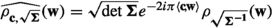, we get

\begin{aligned} \left| 1 - (\sqrt{\det {\textbf{U}}})^{-1}\widehat{\rho _{{\textbf{x}}', \sqrt{{\textbf{U}}}}}({\varLambda }^*)\right| \le \rho _{\sqrt{{\textbf{U}}^{-1}}}({\varLambda }^*\setminus \{0\}) \le \varepsilon . \end{aligned}

For the denominator, we first notice that for two positive semi-definite matrices $${\textbf{A}}$$ and $${\textbf{B}}$$, if $${\textbf{A}}- {\textbf{B}}$$ is positive semi-definite, then $$\rho _{\sqrt{{\textbf{A}}}}({\textbf{w}}) \ge \rho _{\sqrt{{\textbf{B}}}}({\textbf{w}})$$ for all $${\textbf{w}}\in {\mathbb {R}}^n$$. Since $${\textbf{U}}^{-1} - {\textbf{R}}^{-1} = {\textbf{S}}^{-1}$$ is positive semi-definite, it yields $$\rho _{\sqrt{{\textbf{R}}^{-1}}}({\varLambda }^*\setminus \{0\}) \le \rho _{\sqrt{{\textbf{U}}^{-1}}}({\varLambda }^*\setminus \{0\}) \le \varepsilon$$. Therefore, using the same method as above, we get

\begin{aligned} \left| 1 - (\sqrt{\det {\textbf{R}}})^{-1}\widehat{\rho _{-{\textbf{a}}, \sqrt{{\textbf{R}}}}}({\varLambda }^*)\right| \le \rho _{\sqrt{{\textbf{R}}^{-1}}}({\varLambda }^*\setminus \{0\}) \le \varepsilon . \end{aligned}

\begin{aligned} \frac{(\sqrt{\det {\textbf{R}}}\sqrt{\det {\textbf{S}}} / \sqrt{\det {\textbf{T}}})^{-1} \widehat{\rho _{{\textbf{x}}', \sqrt{{\textbf{U}}}}}({\varLambda }^*)}{(\sqrt{\det {\textbf{R}}})^{-1} \widehat{\rho _{-{\textbf{a}}, \sqrt{{\textbf{R}}}}}({\varLambda }^*)} \in \left[ \frac{1-\varepsilon }{1+\varepsilon } , \frac{1+\varepsilon }{1-\varepsilon }\right] \subseteq [1-2\varepsilon , 1+4\varepsilon ], \end{aligned}

assuming that $$\varepsilon < 1/2$$. We thus end up with $$\left| Y({\textbf{x}}) - D_{\sqrt{{\textbf{T}}}}({\textbf{x}})\right| \le 4\varepsilon D_{\sqrt{{\textbf{T}}}}({\textbf{x}})$$. Integration and factor 1/2 of the statistical distance yield the lemma.

$$\square$$

We also need another lemma related to the inner product of $$K_{\mathbb {R}}^d$$ (which results in an element of $$K_{\mathbb {R}}$$) between a discrete Gaussian vector and an arbitrary one. In particular, we use Lemma 2.15 in the proof of Lemma 3.5 in order to decompose a Gaussian noise into an inner product. It generalizes [47, Cor. 3.10] to the module case. A specific instance is proven in the proof of [24, Lem. 4.15], which is later mentioned (without proof) in [50, Lem. 5.5].

### Lemma B.5

([24, Lem. 2.13]) Let $${\textbf{r}}\in ({\mathbb {R}}^+)^n \cap H$$$${\textbf{z}}\in K^d$$ fixed and $${\textbf{e}}\in K_{\mathbb {R}}^d$$ sampled from $$D_{\sqrt{\varvec{{\Sigma }}}}$$, where $$\sqrt{\varvec{{\Sigma }}} = [\delta _{i,j}{\textrm{diag}}({\textbf{r}})]_{i,j \in [d]} \in {\mathbb {R}}^{nd \times nd}$$. Then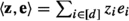is distributed according to $$D_{{\textbf{r}}'}$$ with $$r'_j = r_j\sqrt{\sum _{i \in [d]} \left| \sigma _j(z_i)\right| ^2}$$.

### Proof (of Lemma 2.15)

Consider $${\textbf{h}}\in (K_{\mathbb {R}})^d$$ distributed according to $$D_{{\textbf{r}}', \ldots , {\textbf{r}}'}$$, where $${\textbf{r}}'$$ is given by $$r'_j = \gamma / \sqrt{\sum _{i \in [d]} \left| \sigma _j(z_i)\right| ^2}$$ for $$j \in [n]$$. Then by Lemma B.5,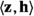is distributed as $$D_\gamma$$ and therefore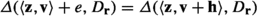. Now, we denote $${\textbf{t}}$$ such that $$t_j = \sqrt{\beta ^2 + (r'_j)^2}$$ for $$j \in [n]$$. Note that by assumption

\begin{aligned} \min _{j \in [n]} \beta r'_j / t_j&= (1/\beta ^2 + \max _{j \in [n]} \sum _{i \in [d]} \left| \sigma _j(z_i)\right| ^2 / \gamma ^2)^{-1/2} \\&= (1/\beta ^2 + \left| \left| {\textbf{z}}\right| \right| _{2, \infty }^2 / \gamma ^2)^{-1/2} \ge \eta _\varepsilon (M). \end{aligned}

Lemma B.4 therefore applies and yields that $${\textbf{v}}+ {\textbf{h}}$$ is distributed as $$D_{{\textbf{t}}, \ldots , {\textbf{t}}}$$, within statistical distance at most $$2\varepsilon$$. By applying once more Lemma B.5 and noticing that the statistical distance does not increase when applying a function (here the inner product with $${\textbf{z}}$$), then we get that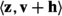is distributed as $$D_{\textbf{r}}$$ within statistical distance at most $$2\varepsilon$$, where $$r_j = t_j\sqrt{\sum _{i \in [d]} \left| \sigma _j(z_i)\right| ^2} = \sqrt{\beta ^2\sum _{i \in [d]} \left| \sigma _j(z_i)\right| ^2 + \gamma ^2}$$ for $$j \in [n]$$. $$\square$$

### Proof

Let $${\mathcal {O}}$$ be an oracle for $$\text {ext-M-LWE}_{n,k,m,q,\psi ,{\mathcal {Z}}}^\ell$$. For each $$i \in \{0, \ldots , \ell \}$$, we denote by $${\mathcal {H}}_i$$ the hybrid distribution defined as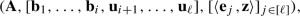where $${\textbf{A}}\sim U(R_q^{m \times k})$$, the $${\textbf{u}}_j$$ are independent and identically distributed (i.i.d.) from $$U((q^{-1}R/R)^m)$$, the $${\textbf{e}}_j$$ are i.i.d. from $$\psi ^m$$, and $${\textbf{b}}_j = q^{-1}{\textbf{A}}{\textbf{s}}_j + {\textbf{e}}_j \bmod R$$ for $${\textbf{s}}_j$$ i.i.d. from $$U(R_q^k)$$ for every $$j \in [\ell ]$$. By definition, we have $${\textrm{Adv}}[{\mathcal {O}}] = |{\mathbb {P}}[{\mathcal {O}}({\mathcal {H}}_\ell )=1] - {\mathbb {P}}[{\mathcal {O}}({\mathcal {H}}_0)=1]|$$. The reduction $${\mathcal {A}}$$ works as follows.

1. 1.

Sample $${\textbf{z}}\hookleftarrow U({\mathcal {Z}})$$ and get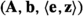as input of $$\text {ext-M-LWE}_{n,k,m,q,\psi ,{\mathcal {Z}}}^1$$.

2. 2.

Sample $$i^* \hookleftarrow U([\ell ])$$.

3. 3.

Sample $${\textbf{s}}_1, \ldots , {\textbf{s}}_{i^* -1} \hookleftarrow U(R_q^k)$$$${\textbf{e}}_1, \ldots , {\textbf{e}}_{i^*-1}, {\textbf{e}}_{i^*+1}, \ldots , {\textbf{e}}_\ell \hookleftarrow \psi ^m$$ and finally $${\textbf{u}}_{i^*+1}, \ldots , {\textbf{u}}_\ell \hookleftarrow U((q^{-1}R/R)^m)$$.

4. 4.

Compute $${\textbf{b}}_j = q^{-1}{\textbf{A}}{\textbf{s}}_j + {\textbf{e}}_j \bmod R$$ for all $$j \in [i^* - 1]$$.

5. 5.

Define the hybrid matrix $${\textbf{B}}= [{\textbf{b}}_1, \dots , {\textbf{b}}_{i^*-1}, {\textbf{b}}, {\textbf{u}}_{i^*+1}, \dots , {\textbf{u}}_\ell ]$$, and the error matrix $${\textbf{E}}= [{\textbf{e}}_1, \ldots , {\textbf{e}}_{i^*-1}, {\textbf{e}}, {\textbf{e}}_{i^*+1}, \ldots , {\textbf{e}}_\ell ]$$. Then call the oracle $${\mathcal {O}}$$ on input $$({\textbf{A}}, {\textbf{B}}, {\textbf{E}}^T{\textbf{z}})$$, and return the same output as $${\mathcal {O}}$$.

If $${\textbf{b}}$$ is uniform, then the distribution in 5. is exactly $${\mathcal {H}}_{i^* -1}$$ whereas if $${\textbf{b}}$$ is $$\text {M-LWE}$$, then the distribution is $${\mathcal {H}}_{i^*}$$. By a standard hybrid argument, the oracle can distinguish between the two for some $$i^*$$ if it can distinguish between $${\mathcal {H}}_0$$ and $${\mathcal {H}}_\ell$$. So the output is correct over the randomness of $$i^*$$. Since $$i^*$$ is uniformly chosen we have

\begin{aligned} {\textrm{Adv}}[{\mathcal {A}}]&= \left| {\mathbb {P}}[{\mathcal {A}}({\textbf{b}}\text { }\text {M-LWE})=1] - {\mathbb {P}}[{\mathcal {A}}({\textbf{b}}\text { uniform})=1]\right| \\&= \left| \sum _{i^* \in [\ell ]} \frac{1}{\ell }{\mathbb {P}}[{\mathcal {A}}({\mathcal {H}}_{i^*})=1] - \sum _{i^* \in [\ell ]} \frac{1}{\ell }{\mathbb {P}}[{\mathcal {A}}({\mathcal {H}}_{i^* -1})=1] \right| \\&= \frac{1}{\ell }{\textrm{Adv}}[{\mathcal {O}}]. \end{aligned}

$$\square$$

### Proof

We start by describing the transformation T of  to move from $$\text {M-LWE}$$ to $$\text {M-ISIS}$$. Given $$({\textbf{A}}, {\textbf{b}}) \in R_q^{m\times d}\times R^m$$, where $${\textbf{A}}$$ is uniformly sampled, T first checks if the rows of $${\textbf{A}}$$ generate $$R_q^d$$. If not, T returns $$\perp$$. By the quantity defined in Section 2.1T aborts at this step with probability $$\delta (m,d)$$ (which can be upper bound from Lemma 2.6). We now condition on $${\textbf{A}}$$ being non-singular. From $${\textbf{A}}$$T computes $${\textbf{B}}\in R_q^{m \times (m-d)}$$ whose columns generate the set of vectors $${\textbf{x}}\in R_q^m$$ that verify $${\textbf{A}}^T{\textbf{x}}= {\textbf{0}} \bmod qR$$T samples $${\textbf{U}}\in R_q^{(m-d)\times (m-d)}$$ uniformly at random such that $${\textbf{U}}$$ is invertible in $$R_q$$, and define $${\textbf{B}}' = {\textbf{B}}{\textbf{U}}$$. As $${\textbf{A}}$$ is uniform in the set of non-singular matrices, $${\textbf{B}}'$$ is uniform in the set of matrices whose rows generate $$R_q^{m-d}$$. Again, by definition of $$\delta (\cdot , \cdot )$$, we get $${\varDelta }({\textbf{B}}', U(R_q^{m \times (m-d)})) \le \delta (m,m-d)$$. Finally, T computes $${\textbf{c}}= {{\textbf{B}}'}^T{\textbf{b}}\bmod qR$$, and returns $$({\textbf{B}}', {\textbf{c}})$$.

Assume that there exists an adversary $${\mathcal {A}}$$ that attacks the $$\varepsilon '$$-uninvertibility of $$\text {M-ISIS}$$. We construct $${\mathcal {B}}$$ that breaks the $$\varepsilon$$-univertibility of $$\text {M-LWE}$$ by calling $${\mathcal {A}}$$ on the sampled transformed by T. Consider $$({\textbf{A}}, {\textbf{A}}{\textbf{s}}+ {\textbf{e}}\bmod qR)$$, with $$({\textbf{s}},{\textbf{e}}) \hookleftarrow U(R_q^d) \times {\mathcal {X}}$$. We denote E the event $$\{{\mathcal {B}}({\textbf{A}}, {\textbf{A}}{\textbf{s}}+{\textbf{e}}+ qR) = ({\textbf{s}},{\textbf{e}})\}$$. Then

\begin{aligned} {\mathbb {P}}[E]&= {\mathbb {P}}[{\textbf{A}}\text { non-singular}]{\mathbb {P}}[E | {\textbf{A}}\text { non-singular}] + {\mathbb {P}}[{\textbf{A}}\text { singular}]\underbrace{{\mathbb {P}}[E | {\textbf{A}}\text { singular}]}_{0\text { (abort)}}\\&= (1 - \delta (m,d)){\mathbb {P}}[{\mathcal {A}}({\textbf{B}}', {\textbf{c}}) = {\textbf{e}}| {\textbf{A}}\text { non-singular}]\\&> (1-\delta (m,d))\cdot (\varepsilon ' - \delta (m,m-d))\\&= \varepsilon . \end{aligned}

Indeed, by the transformation, we have

\begin{aligned} ({\textbf{B}}')^T{\textbf{b}}\bmod qR&= ({\textbf{B}}')^T{\textbf{A}}{\textbf{s}}+ ({\textbf{B}}')^T{\textbf{e}}\bmod qR\\&= ({\textbf{A}}^T{\textbf{B}}' \bmod qR)^T{\textbf{s}}+ ({\textbf{B}}')^T{\textbf{e}}\bmod qR\\&= ({\textbf{B}}')^T{\textbf{e}}\bmod qR. \end{aligned}

Then, $${\mathcal {B}}$$ uses linear algebra to recover $${\textbf{s}}$$ from $${\textbf{b}}- {\textbf{e}}$$. The proof for one-wayness is the same where $$E = \{g_{{\textbf{A}}}({\mathcal {B}}({\textbf{A}}, {\textbf{A}}{\textbf{s}}+ {\textbf{e}}\bmod qR)) = {\textbf{A}}{\textbf{s}}+ {\textbf{e}}\bmod qR\}$$ (recalling that $$g_{{\textbf{A}}}({\textbf{s}}, {\textbf{e}}) = {\textbf{A}}{\textbf{s}}+{\textbf{e}}\bmod qR$$). For the pseudorandomness, we define $$E = \{{\mathcal {B}}({\textbf{A}}, {\textbf{b}}\text { uniform}) = 1\}$$$$E' = \{{\mathcal {B}}({\textbf{A}}, {\textbf{b}}= {\textbf{A}}{\textbf{s}}+{\textbf{e}}\bmod qR) = 1\}$$, and F the event $$\{{\textbf{A}}\text { non singular}\}$$. It then holds that

\begin{aligned}&|{\mathbb {P}}[E] - {\mathbb {P}}[E']| \\&\quad = {\mathbb {P}}[{\textbf{A}}\text { non-singular}]\cdot \left| {\mathbb {P}}[E | {\textbf{A}}\text { non-singular}] - {\mathbb {P}}[E' | {\textbf{A}}\text { non singular}]\right| \\&\quad = (1 - \delta (m,d))\left| {\mathbb {P}}[{\mathcal {A}}({\textbf{B}}', {\textbf{c}}\text { uniform}) = 1 | F] - {\mathbb {P}}[{\mathcal {A}}({\textbf{B}}', ({\textbf{B}}')^T{\textbf{e}}\bmod qR) = 1 | F]\right| \\&\quad > (1-\delta (m,d))\cdot (\varepsilon ' - 2\delta (m,m-d))\\&\quad = \varepsilon , \end{aligned}

concluding the proof. $$\square$$

### Proof

The transformation T now works as follows. Given $$({\textbf{B}}, {\textbf{c}}) \in R_q^{m \times (m-d)}\times R^{m-d}$$ with $${\textbf{B}}$$ uniformly distributed, T checks whether the rows of $${\textbf{B}}$$ generate $$R_q^{m-d}$$. If not, it aborts, and that with probability $$\delta (m,m-d)$$. Conditioning on $${\textbf{B}}$$ being non-singular, T computes $${\textbf{A}}\in R_q^{m \times d}$$ that generate $$\{{\textbf{x}}\in R_q^m$$: $${\textbf{B}}^T{\textbf{x}}= {\textbf{0}} \bmod qR\}$$. The transformation then randomizes $${\textbf{A}}$$ by a random matrix $${\textbf{U}}\in R_q^{d \times d}$$ that is invertible in $$R_q$$ to obtain $${\textbf{A}}' = {\textbf{A}}{\textbf{U}}$$. Similarly as in the previous proof, $${\varDelta }({\textbf{A}}', U(R_q^{m \times d})) \le \delta (m,d)$$. Then, T finds a vector $${\textbf{b}}$$ such that $${\textbf{B}}^T{\textbf{b}}= {\textbf{c}}\bmod qR$$, and returns $$({\textbf{A}}', {\textbf{b}})$$. Note that if $${\textbf{c}}= {\textbf{B}}^T{\textbf{e}}\bmod qR$$ for some $${\textbf{e}}\hookleftarrow {\mathcal {X}}$$, then $${\textbf{b}}- {\textbf{e}}$$ is in the span of the columns of $${\textbf{A}}'$$ and therefore, there exists $${\textbf{s}}\in R_q^d$$ such that $${\textbf{b}}- {\textbf{e}}= {\textbf{A}}'{\textbf{s}}\bmod qR$$. If $${\textbf{c}}$$ is uniform, we can argue that $${\textbf{b}}$$ is also uniform. Using the same calculations as before, we get that

\begin{aligned} \text{ Adv }[{\mathcal {B}}] > (1 - \delta (m, m-d))\cdot (\varepsilon ' - \delta (m,d)) = \varepsilon , \end{aligned}

where $$\text{ Adv }[{\mathcal {B}}]$$ denotes the probability of breaking uninvertibility or one-wayness, or the absolute difference of probability in the case of pseudorandomness. $$\square$$

### Proof

Consider the distribution $${\mathcal {D}}$$ supported over $$R_q^d \times R_q$$ that is either $$A_{{\textbf{s}}, \psi }$$ or $$U(R_q^d \times R_q)$$.

Construction: Sample independently $$(({\textbf{a}}_i, b_i))_{i \in [m']}$$ from $${\mathcal {D}}$$. In both cases, the first component is uniformly distributed over $$R_q^d$$. If there is no subset $$S \subseteq [m']$$ of size d such that the $$({\textbf{a}}_i)_{i \in S}$$ are $$R_q$$-linearly independent, the reduction aborts. By the quantity defined in Section 2.1, this happens with probability $$\delta '(m',d)$$. So now, we assume that there exists a set $$S \subseteq [m']$$ of size d such that the $$({\textbf{a}}_i)_{i \in S}$$ are $$R_q$$-linearly independent. Consider the matrix $${\overline{{\textbf{A}}}} \in R_q^{d \times d}$$ whose rows are the $$({\textbf{a}}_i^T)_{i \in S}$$, and $${\overline{{\textbf{b}}}} \in R_q^d$$ whose coefficients are the $$(b_i)_{i \in S}$$. By construction, $${\overline{{\textbf{A}}}}$$ is invertible in $$R_q^{d \times d}$$. Additionally, if $${\mathcal {D}} = A_{{\textbf{s}}, \psi }$$, then $${\overline{{\textbf{b}}}} = {\overline{{\textbf{A}}}}{\textbf{s}}+ {\textbf{x}}\mod qR^\vee$$ for $${\textbf{x}}$$ sampled from $$\psi ^d$$. On the other hand, if $${\mathcal {D}} = U(R_q^d \times R_q)$$, then $${\overline{{\textbf{b}}}}$$ is uniform over $$R_q^d$$.

Reduction: The transformation T works as follows. Given $$({\textbf{a}}, b)$$ sampled from $${\mathcal {D}}$$ as input:

• Compute $${\textbf{a}}' = -({\overline{{\textbf{A}}}})^{-T}\cdot {\textbf{a}}\mod qR$$;

• Compute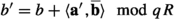;

• Output $$({\textbf{a}}', b')$$.

First, we verify that $$({\textbf{a}}', b')$$ indeed belongs to $$R_q^d \times R_q$$. Since $${\overline{{\textbf{A}}}}$$ is invertible modulo qR, then $$-({\overline{{\textbf{A}}}})^{-T}$$ is in $$R_q^{d \times d}$$. Therefore, $${\textbf{a}}'$$ is also in $$R_q^d$$. Additionally, as $${\overline{{\textbf{b}}}} \in R_q^d$$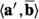is in R. It thus holds that $$b'$$ is in $$R_q$$.

As $$-({\overline{{\textbf{A}}}})^{-T}$$ is invertible modulo qR, and $${\textbf{a}}$$ is uniform in $$R_q^d$$, then $${\textbf{a}}'$$ is also uniform in $$R_q^d$$. Now, we look at the distribution of $$b'$$ in both cases. First, assume that $${\mathcal {D}} = A_{{\textbf{s}}, \psi }$$. Thenfor some  $$e \hookleftarrow \psi$$, and  $${\overline{{\textbf{b}}}} = {\overline{{\textbf{A}}}}{\textbf{s}}+ {\textbf{x}}\mod qR$$. It holds that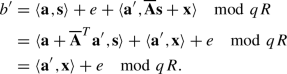So  $$({\textbf{a}}', b')$$ is indeed distributed according to  $$A_{{\textbf{x}}, \psi }$$ for  $${\textbf{x}}\hookleftarrow \psi ^d$$ as desired.

Now assume that  $${\mathcal {D}} = U(R_q^d \times R_q)$$. Then b is uniform over  $$R_q$$ and  $${\overline{{\textbf{b}}}}$$ is uniform over  $$R_q^d$$. So $$b'$$ is clearly uniform over $$R_q$$ as well, proving that $$({\textbf{a}}', b')$$ is uniformly distributed over $$R_q^d \times R_q$$ as desired. $$\square$$

## Rights and permissions

Springer Nature or its licensor (e.g. a society or other partner) holds exclusive rights to this article under a publishing agreement with the author(s) or other rightsholder(s); author self-archiving of the accepted manuscript version of this article is solely governed by the terms of such publishing agreement and applicable law.

Reprints and Permissions